http://people.csail.mit.edu/jaffer/SimRoof/Convection

# Convection From a Rectangular Plate

## Abstract

While there has been much research into convection, A formula which completely models natural, mixed, and forced convection from a rectangular isothermal plate seems to be lacking. Nearly all of the research on convection from plates addresses only plates with a horizontal edge and a smooth surface. My research and experiments on Mixed Convection from a Rough Plate extends the model to rough surfaces. Through the use of effective-characteristic-length, the model is extended to natural convection for all orientations of a plate, not just those having a horizontal edge, off-axis forced (but in-plane) flows, and forced convection averaged over all in-plane directions of flow.

A related model for convection from symmetrical peaked roofs is also developed.

## Introduction

### Motivation

The most important thermal processes for a building's roof are insolation, convection, and thermal radiation. The most complicated to compute is convection because it depends on so many variables: air and surface temperatures, humidity, wind, and roof size, shape, orientation, and (surface) roughness.

Currently, established theory is not sufficient to compute convection from roof parameters and meteorological data. This work rectifies that situation.

### Convective Heat Transfer

Says Wikipedia:

Convective heat transfer, often referred to simply as convection, is the transfer of heat from one place to another by the movement of fluids. Convection is usually the dominant form of heat transfer in liquids and gases.

To model the thermal dynamics of roofs we are interested in the convective flow of heat to or from the top surface of the roof. For a flat plate at a uniform temperature T, the rate of heat flow (in Watts) due to convection from one side of the plate is:

 h ⋅ A ⋅ ΔT h W/(K⋅m2) convective surface conductance A m2 area of one side of plate ΔT K T − Tenv T K plate temperature Tenv K fluid temperature

The complication is that the value of h depends on temperatures, fluid-velocity, and the area, shape, orientation, and roughness of the plate surface. A value of h for a 1 m by 1 m plate will usually be larger (and never smaller) than h for a 2 m by 2 m plate under otherwise identical conditions. The larger plate will transfer more heat because it has four times the area of the smaller plate, but not more than 4 times the heat.

### Types of Convection

Convection is natural (free), forced, or mixed. In natural convection, fluid motion is driven by the difference in temperature between the plate and the fluid. In forced convection, fluid motion is driven by an external force such as wind. Mixed convection is a mixture of natural and forced convection occurring at low fluid speeds.

(Natural) upward convection is produced by an upward-facing plate which is warmer than the fluid, or a downward-facing plate which is colder than the fluid. (Natural) downward convection is produced by an upward-facing plate which is cooler than the fluid, or a downward-facing plate which is warmer than the fluid.

A correlation (approximate equation relating dimensionless quantities) can model an isothermal surface or a constant heat flux through the surface. The correlations for these two regimes usually differ only in coefficients and additive constants. This article addresses convection from isothermal plates.

A forced convection correlation can be for local heat flow or for heat flow averaged across the surface. Section "Laminar-Turbulent Progression" gives the derivation for average heat flow from the local heat flow which appears in several texts. While it produces the customary averaged correlations for purely laminar or purely turbulent flow, the correlation it produces for transitional flows requires a Reynolds-number threshold from measurement of the specific configuration under investigation, which limits its predictive ability.

Convection correlations can be derived from theory, numerical simulation, or experiment (empirical). The accepted correlations for natural convection are empircal.

At the plate surface, the fluid velocity is near zero. At some distance from the plate surface the fluid velocity approaches the bulk fluid velocity. In between is the boundary layer. Flow in the boundary layer is laminar or turbulent. The h values for turbulent regions of the plate (boundary layer) are larger than h values for laminar regions. Natural upward convection (from a horizontal plate) transitions from laminar to turbulent at Rayleigh numbers (Ra) near 107 (Clear et al), placing it between the ranges for correlations T9.7 and T9.8 (which intersect at 4.7×106). Convection from a vertical plate has a transition around Gr=Ra/Pr=109.

Natural convection is strongly affected by the inclination of a plate. The natural convective surface conductivity from a vertical plate is between the conductivities of upward facing and downward facing level plates of the same size. Upward convection has the largest heat flow. Forced convection is insensitive to inclination.

Forced convection is greater for rough surfaces than for smooth ones. Natural convection is insensitive to roughness whose mean height is much less than the dimensions of the plate.

## 1997 ASHRAE Fundamentals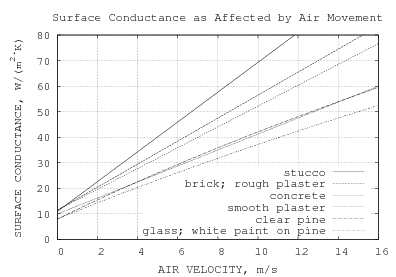The 1997 ASHRAE Fundamentals Handbook (SI) takes an engineering approach to convective heat transfer. Fig. 24.1 (reconstructed to the right) has a graph of surface conductance h as a function of wind velocity V for 6 building materials. That conductance is for a ΔT of 5.5°C at a mean temperature of −6.7°C and includes blackbody emissions, which add a constant amount to each curve. About Fig. 24.1, the Handbook also states:

Other tests on smooth surfaces show that the average value of the convection part of the surface conductance decreases as the length of the surface increases.

The graph is based on  which gives the dimensions of the plate as a 0.305 m square. Without a quantitative relation between surface conductance and plate size, application to surfaces as large as roofs is uncertain.

Two of the lines are bent: "Clear pine" and "Glass and white paint on pine". The formulas I fit for glass and pine are:

 Glass Clear Pine h(V) = (b2 − 4 a c + 4 a V)1/2 − b 2 a hg hp a = 1/882 a = 4/3999 b = 257/882 b = 973/3999 c = −1060/441 c = −2680/1333

### Thermal Emissivity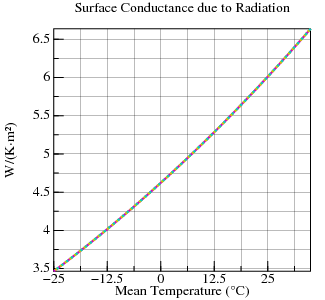The first task is to separate the surface conductivity due to convection from that due to radiative transfer. Measurements of convective surface conductance take place in a large enclosure whose temperature matches the fluid (air) inside it. To the right is plotted the difference in blackbody radiance between the plate and enclosure divided by the temperature difference. Plots for temperature differences of 1°C, 5°C, and 25°C are superimposed and look identical at this scale.

The maximum surface conductivity due to radiation at −6.7°C±2.75°C is 4.29 W/(K⋅m2). If the surface and test apparatus both have emissivity of 0.95, it is 3.87 W/(K⋅m2). But the suite of materials tested do not necessarily have the same emissivity.

There are multiple books and web-pages offering tables of emissivity for building materials. Of the five excerpted below, those that cite a source cite handbooks or textbooks, so they are not original data.  gives emissivities at specified temperatures. None of these five sources provided a value for stucco.

source citing
[E1] EMISSIVITY EXPLAINED IN LAYMAN'S TERMS,
Fig 1.2 EMISSIVITY OF BUILDING MATERIALS,
Horizon Energy Systems.
Handbook of Chemistry
 A Heat Transfer Textbook, Third Edition [PDF],
by John H. Lienhard IV and John H. Lienhard V,,
Cambridge, MA, Phlogiston Press, 2008
[E3] Emissivity Coefficients of some common Materials,
The Engineering Toolbox
[E4] Emissivity Table,
ThermoWorks Inc., 2012
 2009 ASHRAE Fundamentals Handbook (SI)
American Society of Heating Refrigerating and Air-conditioning Engineers Inc.
ISBN: 978-1-931862-70-7 (I-P); 978-1-931862-71-4 (SI)
ISSN: 1523-7222 (I-P); 1523-7230 (SI)
Mills, A.F., 1999.,
Basic heat and mass transfer.

glass paint wood plaster plaster brick concrete
[E1] .94 .94 avg 16 colors .95 .91 rough coat .92 common red .95
 .94 smooth .92-.96 var. oils .90 oak planed .92 rough lime .93 red rough .94 rough
[E3] .92-.94 smooth .96 .95 pine .98 .91 rough .93 red rough .94 rough
[E4] .92 .97 oil, grey, flat .90 planed .86-.90 .91 rough coat .93 common red .92
 .91 smooth .90 white acrylic .89 rough .90 .91 rough
E1 - E4 consensus values:
.93 .95 .92 .91 .93 .94

Source  gives emissivities which are less than those given by the other sources; so I didn't use it in finding consensus values. For six of the seven materials the spread in emissivity values is small enough for consensus values. Although non-metallic paints are given emissivity values from 0.80 to 1.00, both 0.80 values are for enamel; the 1.00 value is only for black velvet coating 9560 series optical black, neither of which was used in the 1930 experiments.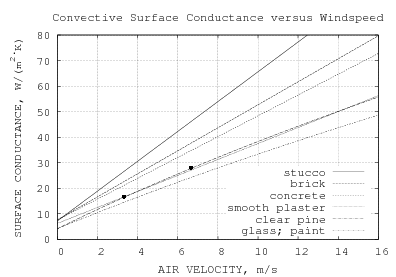In the figure to the right I have subtracted out the radiative conductances as moderated by the consensus emissivities and painted apparatus (ε=.95). The emissivity for plaster (.91) is used for stucco. Thus this is a plot of surface conductivities due only to convection, ie. ε=0.0.

Table 1 of chapter 24 gives two numbers for convection in moving air (any position) of a surface with emissivity ε=.9 at 21°C, but without specifying the surface material. These two points, with radiative conductance subtracted out, are marked with dots on the graph. They are closest to the curve for clear pine.

### Still Air

At V=0, the surface conductance is due to natural, not forced, convection.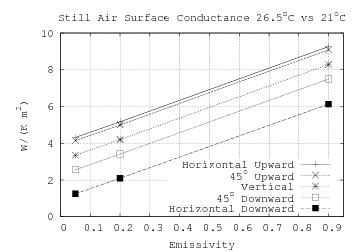Table 24.1 gives still-air conductance for flat plates in five positions with emissivities .90, .20, and .05 which, being collinear (chart at right), allow the still-air conductances to be extrapolated to zero emissivity, h0.0.

Table 1 Surface Conductivity W/(m2⋅K)
θ orientation heat-flow h0.0
−90° horizontal up 4.03
−45° inclined up 3.86
vertical up 3.06
+45° inclined down 2.27
+90° horizontal down 0.96

Surface Conductances as Affected by Air Velocity, Temperature and Character of Surface by F.B. Rowley, A.B. Algren, and J.L. Blackshaw is the source for Fig. 24.1. Their test plate was inserted (flush with interior) into a .305 m by .305 m opening cut from one vertical side of a .152 m by .305 m rectangular duct over 7 m long.

Rowley at al take and report measurements with the flow shut off. In such a long duct with no forced airflow, equilibrium must involve conduction through the walls of the (insulated) duct. The equilibrium temperature range they report for 0 m/s flow is 9°C to 40°C, while for forced flow the equilibrium temperatures range is −16°C to 31°C. Fig. 24.1 specifies a temperature of −6.7°C; so they must have extrapolated the 0 m/s values.

Convective Surface Conductance h (Theory)
ΔTMean Temperature
10°C50°C90°C
10 K3.66 W/(m2⋅K)3.43 W/(m2⋅K)3.25 W/(m2⋅K)
20 K4.49 W/(m2⋅K)4.20 W/(m2⋅K)3.97 W/(m2⋅K)
30 K5.06 W/(m2⋅K)4.73 W/(m2⋅K)4.47 W/(m2⋅K)

As shown to the right, natural convective surface conductance is much more sensitive to temperature difference than mean temperature (average of surface and air temperatures); but only mean temperatures are revealed in their graphs. The mean temperature in this case uses the air temperature 25 mm from the center of the surface.

Table 1. Test Data for Smooth Plaster Surface
Temperature
Test Test
Surface
25 mm
From Test
Surface
Mean Difference Surface
Conductance
257 30.3°C 1.6°C 15.9°C 28.7 K 10.90 W/(m2⋅K)
264 52.3°C 26.9°C 39.6°C 25.4 K 11.48 W/(m2⋅K)

Table 1 Test Data for Smooth Plaster Surface shows one page of their measurements. Two of its rows are for still air. Here they are converted to metric units. The surface conductance column includes both the convective and radiative surface conductances. The radiative surface conductance depends on the inner wall temperature of the duct, which is not provided. Rowley et al write:

In order to obtain average radiation conditions, the inside surface of the test duct was painted a dull gray, and all of the pipe outside the refrigerator was covered with a one-inch [25 mm] thick blanket insulating material. With this arrangement, the surfaces immediately around the test surface were at substantially the same temperature as the surrounding air, practically the same as that for the average wall...
Preliminary tests had shown that when the thermocouple was placed in contact with the test surface and gradually moved away from it, the temperature steadily dropped until the couple was about 1/2-in [13 mm]. from the surface, after which this temperature remained uniform and equal to the air temperature, regardless of distance.

The air temperature they refer to is that of the refrigerated room connected through more than 5 m of level duct. It seems clear from the article that the "preliminary tests" were conducted with forced air, calling into question whether the duct inner surface temperature is the same as the air temperature 25 mm from the plate center when flow is unforced.

So how would convection from a vertical plate in a horizontal duct compare with convection from an open-air vertical plate? Inside the duct heated rising air is obstructed and so accumulates in the duct. This reduces the heat transfer from the heated plate, but also raises the temperature of the air which is 25 mm from the plate. When the heat transfer is divided by ΔT, it increases the reported h values.

With processes driving h in opposite directions, these still-air measurements can be justified as neither upper nor lower bounds for natural convective surface conductance. Particularly disappointing is that the relationship between sub-millimeter surface roughness and natural convection from a flat vertical plate remains unquantified (the lack of measured emissivities also contributes uncertainty).

## 2009 ASHRAE Fundamentals

The 2009 ASHRAE Fundamentals Handbook (SI) takes an entirely different approach, characterizing convective heat-transfer using correlations (equations) of dimensionless quantities. Surface roughness is treated only for forced convection inside pipes and ducts. For external plates only horizontal and vertical orientations are covered. It has no example calculations of unobstructed convection from a plate.

It appears that with the move to a more theoretical treatment, 2009 Fundamentals lost practical applicability to roofs. The intent of this article is to develop a theory sufficient to predict convection from rough inclined rectangular roofs.

 Formulas not leading to the final derivations have a gray background.

### Physical Parameters

symbol units description TF K Fluid (Air) Temperature TS K Plate Surface Temperature T K Mean Temperature (TF+TS)/2 P Pa Fluid (Atmospheric) Pressure V m/s Fluid Velocity Rair J/(kg⋅K) Gas Constant for Dry Air = 287.058 J/(kg⋅K) Φ Pa/Pa Relative Humidity

From these parameters are derived density ρ, thermal conductivity k, specific heat cp, and viscosity μ. For dry air, Kadoya, Matsunaga, and Nagashima is the authoritative source for viscosity and thermal conductivity.

The example calculations in this text were performed before the model incorporated humidity. Calculations with a relative humidity of 41% at sea level match the example calculations within 2%.

symbol forced natural formula units description cp ✓ ✓ [see text] J/(kg⋅K) specific heat at constant pressure ρ ✓ ✓ P/(Rair ⋅ T) kg/m3 density k ✓ ✓ [see text] W/(m⋅K) thermal conductivity μ ✓ ✓ [see text] Pa⋅s dynamic viscosity ν ✓ ✓ μ/ρ m2/s kinematic viscosity α ✓ k/(ρ⋅cp) m2/s thermal diffusivity β ✓ 1/T K−1 coefficient of thermal expansion

The moist air values of these properties are computed by combining the values for dry air and water vapor in proportion to their presence in the moist air mixture, in some cases with correction factors. What is true of all the mixture formulas is that at 0% relative humidity the mixture values are identical with the dry air values and at 100% relative humidity at 100°C the mixture values are identical with the steam (water vapor) values.

These mixture formulas come from Tsilingiris and Morvay and Gvozdenac (not ASHRAE). Both sources contain errors; the obvious errors don't occur in the corresponding quantities. Wexler is the authoritative source for water-vapor (partial) pressure versus temperature.

### Thermodynamic and Transport Properties of AirAt 100% relative humidity, the pressure and mass fraction of vapor increase with temperature, but remain less than 10% at 45°C. So humidity will not be a major influence on air's properties at outdoor temperatures.

Model moist air as a mixture of ideal gases. The relative-humidity, Φ, is the ratio of the partial-pressure of water vapor to Psat. Psat is the partial pressure of saturated water vapor at temperature TF (Kelvins):

 Psat = 610.78 ⋅ 107.5 (TF−273.15)/(TF−35.85)

Over the temperature range of interest, this formula for Psat is well within 1% of the values returned by formula 16b in Wexler:

 Psat = exp( −0.63536311×104/TF +0.3404926034×102 −0.19509874×10−1⋅TF +0.12811805×10−4⋅TF2 )

Density ρ, thermal conductivity k, specific heat cp, and viscosity μ are computed at temperature T, the average of TF and TS. But Psat must be evaluated at the bulk fluid temperature TF because the amount of water-vapor in the fluid doesn't change when heated to intermediate temperature T. If TS is colder than TF, then condensation may occur.

Simulation of roofs can side-step the issue by constraining the roof temperature to not drop below the ambient dew-point temperature. Because of water's high latent heat, this treatment should not result in large errors.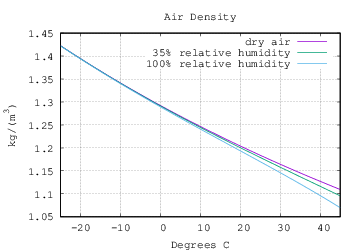For an ideal gas, density ρ is:

 ρ=P M/(R T) R=8.314 J/(kg⋅mol)

Model moist air as a mixture of dry air and water vapor:

 ρ = (P − Φ Psat) Ma + Φ Psat Mv R T R = 8.314 J/(kg⋅mol) Ma = 0.028964 kg/mol Mv = 0.018016 kg/mol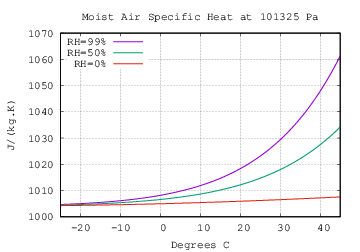The specific heat at constant pressure cp for dry air and water vapor each vary little over our temperature range. But the mixture at a given relative humidity is sensitive to temperature. cpa and cpv are the specific heat of air and water-vapor, respectively. These formulas from Tsilingiris take temperature t in degrees Celsius. xv(T) takes temperature in Kelvins.

 cpa(t) = 1034 −.2849⋅t +.7817×10−3⋅t2 −.4971×10−6⋅t3 +.1077×10−9⋅t4 cpv(t) = 1869−2.578×10−1⋅t +1.941×10−2⋅t2
 cp= cpa(t) (1−xv) Ma + cpv(t) xv Mv (1−xv) Ma + xv Mv xv(T) = Φ Psat(T)/P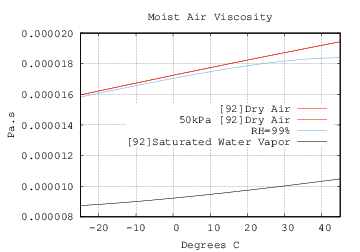Both  and  give formulas for the viscosity of dry air.  matches the data from  better; the values from  are about 1% lower; but result in (lower) Pr values closer to those from many sources. The formula from  is:

 μa = −9.8601×10−7 +9.080125×10−8⋅T −1.17635575×10−10⋅T2 +1.2349703×10−13⋅T3 −5.7971299×10−17⋅T4

The formula from  is:

 μa = −0.40401×10−6 +0.074582×10−6⋅T −5.7171×10−11⋅T2 +2.9928×10−14⋅T3 −6.2524×10−18⋅T4

A line for the viscosity at half air pressure (P=50 kPa) overlays Dry air; so air pressure variations don't significantly affect viscosity at roof conditions.

The viscosity of saturated water vapor is less studied. Tsilingiris gives a formula which returns a viscosity value for 100 C around half of that shown in his own graph of viscosity! Morvay and Gvozdenac give a formula for the viscosity of water vapor which matches Tsilingiris' graph well. With γ=647.27/T:

 μv = γ−1/2 018158.3 + 017762.4 γ + 010528.7 γ2 −003674.4 γ3

Over the temperature range of interest, μv is hardly different from:

 μv = 9.2173×10−6 Pa⋅s + (T − 273.15 K) ⋅ 25.713×10−9 Pa⋅s/K

Morvay and Gvozdenac introduce a parameter they call absolute humidity, the ratio of masses of water vapor and dry air:

 χ= Mv Psat Ma (P−Psat)

The dynamic viscosity of the moist air mixture is:

 μ = μa 1 + ΦAV ⋅ χ + μv 1 + ΦVA / χ

The terms ΦAV and ΦVA are complicated expressions having values ranging from 1.064 to 1.073 and .93 to .923 respectively over the roof range of interest (-25°C to 45°C).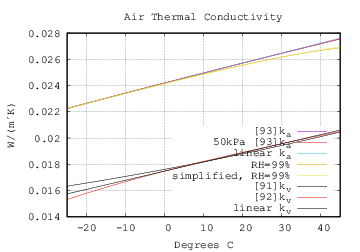The authoritative formula for the thermal-conductivity of dry air is from Kadoya, Matsunaga, and Nagashima:

 ρr = P / (287.058 ⋅ 314.3 ⋅ T) Tr = T / 132.5 ka = 0.0259778 ⋅ ( 0.239503⋅Tr +0.00649768⋅Tr1/2 +1.0 −1.92615⋅Tr−1 +2.00383⋅Tr−2 −1.07553⋅Tr−3 +0.229414⋅Tr−4 +0.402287⋅ρr +0.356603⋅ρr2 −0.163159⋅ρr3 +0.138059⋅ρr4 −0.0201725⋅ρr5 )

A line for the k at half air pressure (P=50 kPa) overlays Dry air on the graph; so air pressure variations don't significantly affect k at roof conditions. Over the roof range of interest this is hardly different from:

 ka = 0.02241 W/(m⋅K) + (T−250 K) ⋅ 76.46×10−6 W/(m⋅K2)

Tsilingiris gives a formula for the thermal conductivity of water vapor:

 kv = 1.761758242×101 +5.558941059×102 T +1.663336663×104 T2

Morvay and Gvozdenac also give a formula for the thermal conductivity of water vapor:

 kv = 1.74822×10-2 +7.69127×10-5 t −3.23464×10-7 t2 +2.59524×10-9 t3 −3.17650×10-12 t4

They diverge mostly at the dry end of the curve, where kv has insignificant effect on the moist air thermal-conductivity (km). Over the roof range of interest the latter curve is hardly different from:

 kv = 0.0174822 W/(m⋅K) + (T−273.15 K) ⋅ 69.4587305×10−06 W/(m⋅K2)

Morvay and Gvozdenac's formula for moist air thermal conductivity is like the formula for viscosity, but with more complicated expressions for ΦAV and ΦVA. Tsilingiris's formula uses the same ΦAV and ΦAV as the viscosity formula:

 k = ka 1 + ΦAV ⋅ χ + kv 1 + ΦVA / χ

The simplified 99% RH curve, which is nearly identical to the 99% RH curve, uses the linear ka and kv models and substitutes 1.07 and 0.93 for ΦAV and ΦVA.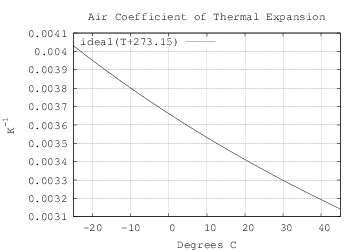For an ideal gas with pressure held constant, the volumetric thermal expansivity (i.e. relative change in volume due to temperature change) is the inverse of temperature. For natural convection from a horizontal plate it is:

β=1/T

For natural convection from a vertical plate (T9.2, T9.3) it is:

β=1/TF

### Characteristic Length

For a flat rectangular plate, Lc is the length of the side parallel to the direction of flow.

For a flat plate, L* is the area (of one side) of the plate divided by its perimeter. Here are formulas for L* of four shapes. l is the length; w is the width; D is the diameter.

 rectangle square infinite strip circular disk L*(w, l) = w⋅l 2 (w+l) L*(w, w) = w 4 L*(w, ∞) = w 2 π D2 / 4 π D = D4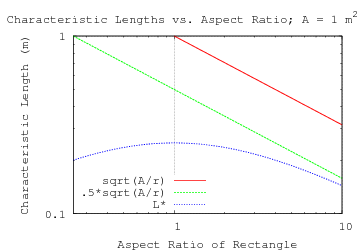It is interesting that a square and the maximal circle inscribed within it have the same L*.

The plot to the right shows how the length of a side (sqrt(A/r)) and L* (area/perimeter) vary with aspect-ratio for a rectangular plate with an area of 1 m2. As the aspect-ratio r grows, L* tends to sqrt(A/r)/2.

The hydraulic-diameter, used as the characteristic length DH in ducts, is related to L*, being 4 times the cross-section area divided by the cross-section perimeter.

### Dimensionless Quantities

Below are formulas for dimensionless quantities governing convection along with ranges for air under the conditions:

Dry-Bulb Temperature
between −25°C and +45°C
Temperature difference between the roof and dry-bulb
between −10 K and +50 K
Atmospheric Pressure
between 88300 Pa and 103600 Pa
Wind Speed
between 1 m/s and 15 m/s
Characteristic Length Lc
between .5 m and 50 m height for a vertically oriented plate;
between .5 m and 50 m length for forced convection;
an area-to-perimeter ratio between 0.25 m and 12 m for natural convection from a horizontally oriented plate;
Mean Height of Roughness ε
between 0 mm and 2 mm.
gravitational acceleration g
9.80665 m/s2

Ranges for vertical plates, where Lc is the height, are marked with a subscripted V. Ranges for horizontal plates, where Lc is the ratio of area to perimeter, are marked with a subscripted H. The Prandtl number is insensitive to Lc, depending only on fluid (air) properties. The Lc for Reynolds and Nusselt numbers in forced convection is the length of the plate in the direction of flow.

Both Gr and Ra have factors of |ΔT|; they will be zero when ΔT is zero. In order to get an idea of the dynamic range of Gr and Ra, the minimum ΔT used for range-of-interest is 1 K rather than 0 K. Re will be zero when the wind-speed is zero. In order to get an idea of the dynamic range of Re, the minimum V used for range-of-interest is 1 m/s rather than 0 m/s.

In convection calculations, Nu is computed from the other dimensionless quantities; then solved for h, the surface conductance (having units W/(m2⋅K)).

symbol formula expansion roof range of interest description Pr ν/α cp⋅μ/k 0.719≤Pr≤0.735 Prandtl number Gr β⋅|ΔT|⋅g⋅Lc3ν2 |ΔT|⋅g⋅Lc3⋅ρ2T⋅μ2 9.35×107≤GrV≤2.02×1015 1.17×107≤GrH≤2.79×1013 Grashof number for natural convection Ra Gr⋅Pr |ΔT|⋅g⋅Lc3⋅ρ2⋅cpT⋅μ⋅k 6.72×107≤RaV≤1.49×1015 8.40×106≤RaH≤2.05×1013 Rayleigh number for natural convection Re V⋅Lc/ν V⋅Lc⋅ρ/μ 2.46×104≤Re≤6.79×107 Reynolds number for forced convection Nu RSTT8.9, T8.11 (forced rough)(forced smooth) 1.08×102≤Nu≤6.13×104 1.08×102≤Nu≤2.62×105 Nusselt number HHT*T9.3 (natural) 33.4≤NuH≤3.63×103 54.4≤NuV≤1.22×104 h Nu⋅k/Lc (forced rough)(forced smooth) 2.38≤h≤147. 2.38≤h≤68.4 convective surface conductance W/(m2⋅K) Nu⋅k/L* Nu⋅k/Lc (natural) 3.02≤hH≤7.58 2.43≤hV≤6.18

It is worth noting that a larger h value doesn't necessarily correspond to a larger Nu value because Nu gets divided by different L values.

## Natural Convection

The table below excerpts those sections of Table 9 from chapter 4 of 2009 ASHRAE Fundamentals Handbook (SI) which deal with natural convection from isothermal (uniform ts) flat plates. HHT* is my generalization of T9.5 through T9.8.

 II. Vertical plate Properties at (ts+t∞)/2 except β at t∞. L=height 0.1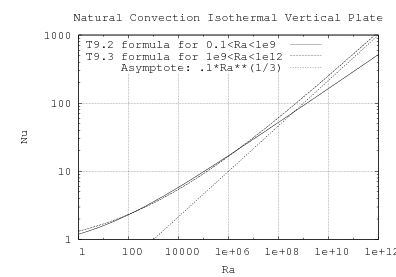### Vertical Plate

The range for T9.2 is supposed to cross over to T9.3 at Ra=109, but the two curves don't match at Ra=109. About T9.3, Churchill and Chu (1975a) conclude that:

Equation (9) [T9.3] based on the model of Churchill and Usagi provides a good representation for the mean heat transfer for free convection from an isothermal vertical plate over a complete range of Ra and Pr from 0 to ∞ even though it fails to indicate a discrete transition from laminar to turbulent flow.

Roofs aren't vertical. But this formula is a component for modeling the cases of roofs which are neither horizontal nor vertical.

The stipulation: "Properties at (ts+t)/2 except β at t." is not in Churchill and Chu . On this subject their paper says only:

For large temperature differences such that the physical properties vary significantly, Ede  recommends that the physical properties be evaluated at the mean of the surface and the bulk temperature. Wylie  provides more detailed theoretical guidance for the laminar boundary-layer regime.

The maximum relative error for Nu in T9.3 if β is 1/t instead of 2/(ts+t) is

 ( ts+t∞ 2 t∞ ) 1/3 −1 = ( Δt 2 t∞ +1 ) 1/3 −1

At t=20°C and ts=70°C the maximum error is less than 3%.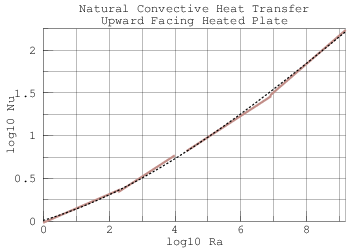### Horizontal Plate

#### Upward Convection

Formulas T9.5 through T9.8 give the horizontal hot top correlations in 4 segments with a gap between 104 and 2.2×104. A function (dashed line) constructed like T9.3 shows good agreement and bridges the gap:

 Nu* range {0.671 + 0.370 Ra1/6}2 1

#### T9.9

Despite being cited (in footnote b), there is no support for T9.9 in either Lloyd and Moran (1974) or Goldstein et al. (1973), which both state "The present results apply to either the heated upward facing heat transfer surface or the cooled downward facing heat transfer surface." Brucker and Majdalani (2005) mis-attribute T9.9 to Lloyd and Moran (1974). Whitaker (2005)  also perpetuates T9.9.

With the Lc values which generate curves matching Fig. 24.1, T9.9 yields h values much larger than Table 24.1.

#### Downward Convection

In Natural convection heat transfer below downward facing horizontal surfaces , Schulenberg develops correlations for downward convection for an infinite strip and circular plate (I added the equivalent T9.2-style expressions):

 plate-shape Schulenberg T9.2-style infinite strip Nu = 0.571 Ra1/5 ⋅ Pr1/5 [1+1.156 Pr3/5]1/3 = 0.544 Ra1/5 [1+(0.785/Pr)3/5]1/3 (45) circular disk Nu = 0.705 Ra1/5 ⋅ Pr1/5 [1+1.48 Pr3/5]1/3 = 0.619 Ra1/5 [1+(0.520/Pr)3/5]1/3 (47)

In Schulenberg's derivation, the characteristic-length used for computing the Rayleigh-number (Ra) is R:

 R half-width of the infinite strip or radius of the circular plate
R for formula (45) is half of the strip width, which is the same as L*.
R for formula (47) is the radius of the disk, which is twice L*.

### Inclined Plate

The formula for convective surface conductance (h) for a heated upward-facing or a cooled downward-facing LH by LW rectangular plate inclined θ from vertical around a level LW edge, 90° (horizontal) to 0°(vertical).

 L* = LH⋅LW / (2 (LH+LW)) h = k⋅ max( NuHHT*(Ra(L*)⋅|sin θ|) / L*, Nu9.3(Ra(LH)⋅cos θ) / LH ) −90°≤θ≤0° UHT3

The formula for convective surface conductance (h) for a heated downward-facing or a cooled upward-facing LH by LW rectangular plate inclined θ from vertical around a level LW edge, 90° (horizontal) to 0°(vertical). Because the derivative of h45* is monotonically decreasing as a function of L, its two terms are collapsed to one term dependent on min(LH, LW).

 R = min(LH, LW)/2 h = k⋅max(Nu9.3(Ra(LH) cos θ)/LH , Nu45(Ra(R) sin θ)/R) 0°≤θ≤+90° UCT3

The sin θ factor is included in order to make the formula continuous with T9.3 at θ=0. It has negligible effect near θ=90°

In A heat transfer textbook, Lienhard and Lienhard attribute the use of g⋅cos θ for inclined laminar convection to:

B. R. Rich.
An investigation of heat transfer from an inclined flat plate in free convection.
Trans. ASME, 75:489–499, 1953.

They attribute the use of g⋅cos θ and g⋅sin θ for inclined turbulent convection to:

G. D. Raithby and K. G. T. Hollands.
Natural convection.
In W. M. Rohsenow, J. P. Hartnett, and Y. I. Cho, editors,
Handbook of Heat Transfer, chapter 4.
McGraw-Hill, New York, 3rd edition, 1998.

### Natural Convective Surface Conductance

Here are the conditions for the Table 1 of chapter 24 still-air convection points, which are plotted in the lower right graph.

symbol value units or L description T 296.9 K mean of plate and ambient temperature ΔT 5.5 K temperature difference between plate and ambient P 101325 Pa air pressure (assumed, not specified) μ 1.86×10-5 Pa⋅s dynamic viscosity k 0.0260 W/(m⋅K) thermal conductivity of air ρ 1.19 kg/m3 air density Pr 0.723 Prandtl number Ra 8.43×106 LH = 0.25 m Rayleigh numbers of smooth 0.25 m square plate Ra* 1.32×105 L* = 0.0625 m Ra 2.31×107 LH = 0.35 m Rayleigh numbers of smooth 0.35 m square plate Ra* 3.61×105 L* = 0.0875 m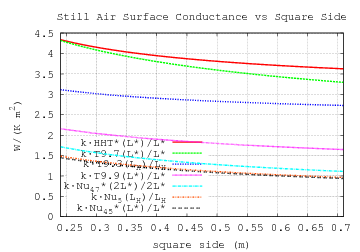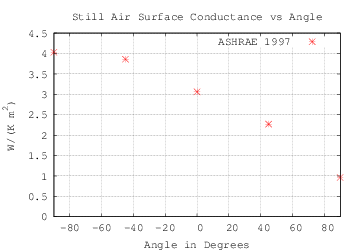The shape and size of the plate are not specified by Table 24.1. The graph to the left shows what the various correlations predict for a smooth square plate with side length from 0.24 m to 0.71 m. The top two traces are for horizontal upward convection using composite correlation HHT* and Table 9 correlation T9.7 respectively. The middle trace is for a vertical plate (hT9.3). The bottom three traces are for a horizontal downward facing hot plate or upward facing cold plate. As discussed above, hT9.9 appears to have no basis in theory or published measurement; it is over 50% larger than surface conductances based on Fujii and Imura (Nu5) and Schulenberg (Nu45).

The hHHT* curve matches the Table 24.1 −90° value at a square-side length of 0.35 m, while the hT9.3 curve matches the Table 24.1 vertical value at a square-side length of 0.37 m. The Nu45 and Nu5 curves match the +90° value around 0.7 m. The upper left plot shows that all of the conductances grow with decreasing length of the square's side; so simply adjusting the square's dimensions will not improve the fit.

Would non-square rectangular plates bring these points closer to their targets? We are looking to reduce h45* while not reducing hHHT* and hT9.3. For the vertical plate, the width does not affect its surface conductance hT9.3. So lets leave the height fixed and adjust the width. The conductance h45* uses the length of whichever side is shorter. So h45* does not change with increasing width. And h45* increases with decreasing width, which degrades the match. Therefore downward facing surface conductance cannot be reduced by changing the aspect-ratio from 1 (square).

From this, we can conclude that the plate-under-test in Table 24.1 was most likely square. In addition to coming within a few percent of the Table 24.1 values, a 0.35 m square is a practical size for measuring convection, requiring an enclosure no more than a few cubic meters in size. For the rest of this article, I will assume that the Table 24.1 plate was a 0.35 m square.

LH=LW=0.35 m; L*=87.5 mm
θ h calculated Table 24.1 relative error
−90°hHHT*4.0374.03+0.2%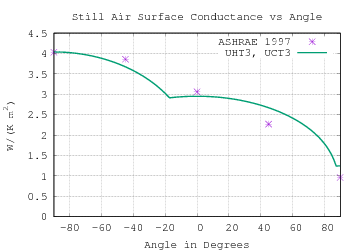−45°hUHT33.6723.86−4.9%
hT9.32.9523.06−3.5%
+45°hUCT32.6712.27+17.7%
+90°hT9.9*1.9650.96+105%
+90°h45*1.2450.96+30.0%

The plot of surface conductance is linked to a larger, high-resolution version. The UHT3 curves applies from −90° to 0°; the UCT3 curve applies from 0° to +90°.

UHT3 uses whichever mode has higher convective heat transfer, and comes within 5% of the Table 24.1 value at −45°.

Small tilts around −90° should not change h much. And that is the behavior of hUHT3. UCT3 exceeds the Table 24.1 value by more than 16% at +45° (inclined facing down). The downward convection from a level plate exceeding the Table 24.1 value by more than 30% is unfortunate, but the natural convection from horizonatal and vertical plates is established by theory and experiment from multiple sources. My paper Mixed Convection from a Rough Plate plots new measurements against UHT3/UCT3 and finds agreement within 10%.

The asymmetry of the curve near vertical (0°) is interesting. As fluid heated at the bottom of a vertical plate rises, it stays near the plate, creating a thermal boundary layer which is wider at the top of the plate than at the bottom. Fig.14(a) from Fujii and Imura(1972) shows that the hydraulic boundary layer is also thicker at the top than the bottom of heated vertical plate.

As the plate tilts up, buoyancy of heated fluid pulls parcels away from the plate, increasing convective transport and surface conductance. Further upward-tilting promotes more (HHT*) vertical mixing at the expense of (T9.3) fluid flowing the length of the plate.

Because the layer of heated fluid is thick at the upper end of a vertical plate, as the plate is tilted downward, the further thickening of the boundary layer does not much affect convection. Further downward-tilting reduces the buoyancy of fluid parcels traveling along the plate.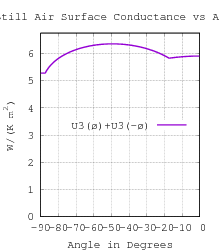### Double-Sided Natural Convection

A correspondent wonders what the convection from both sides of a solar-cell panel is. For natural convection, the double-sided panel shows much less variation with angle than the single-sided case.

### Natural Convection Summary

Natural convection correlations for inclined rectangular plates involve characteristic-lengths derived from LH and LW. The derivation of Leff is in the section Natural Off-Axis Convection Each correlation is computed using its own characteristic-length.

Proposed is the complete natural convective surface conductance for one side of a flat LH by LW rectangular isothermal plate rotated φ around the center of the plate (in its plane) and inclined θ from vertical.

 L* = LH⋅LW / (2 (LH+LW)) R = min(LH, LW)/2 Leff = LH LW |cos φ| LW + |sin φ| LH LVRR Pr = cp⋅μ/k Ra(L) = |ΔT|⋅g⋅ρ2⋅cp⋅L3 / (T⋅μ⋅k) NuHHT*(Ra*) = {0.671 + 0.370 Ra*1/6}2 1≤Ra*≤1012 θ=−90° T9.3(Ra) = {.825 + .387 Ra1/6 [1+(.492/Pr)9/16]8/27 }2 1≤Ra≤1012 θ=0° Nu45(Ra) = 0.544 Ra1/5 [1+(0.785/Pr)3/5]1/3 θ=+90°

 h = k⋅ max( Nu45(Ra(R) sin θ) R , Nu9.3(Ra(Leff) cos θ) Leff ) 0°≤θ≤+90° UCT3E h = k⋅max( NuHHT*(Ra(L*) |sin θ|) L* , Nu9.3(Ra(Leff) cos θ) Leff ) −90°≤θ≤0° UHT3E

These equations assume that T9.3 and Nu45 are independent of surface roughness (with mean-height-of-roughness much smaller than the characteristic length). Evidence from Clear et al supports the independence of HHT* from surface roughness. The section "Natural Convection from a Rough Plate" addresses the effects of surface roughness on T9.3 and Nu45.

## Forced Convection

For a flat plate there is much less experimental data available for forced convection than for natural convection. To accurately measure forced convection requires all the equipment necessary for measuring natural convection incorporated in a very uniform, laminar wind-tunnel. Wind speed and direction add two independent variables, multiplying the number of measurements which must be taken.

The common correlations for forced convection are due to Blasius' 1908 mathematical analysis.

Recalling Dimensionless Quantities, we introduce local versions of Nu and Re:

symbol formula expansion description Nux hx⋅x/k local Nusselt number Nu h⋅Lc/k average Pr ν/α cp⋅μ/k fluid Prandtl number Rex V⋅x/ν V⋅x⋅ρ/μ local Reynolds number Re V⋅Lc/ν V⋅Lc⋅ρ/μ average

The table below excerpts those sections of Table 8 from chapter 4 of 2009 ASHRAE Fundamentals Handbook (SI) which deal with convection from flat plates. The plate orientation is not specified. L is the length of the plate [assumed in the direction of fluid flow]. No citations are given for these 5 correlations. Rec does not appear in the text.

 III. External Flows for Flat Plate: Characteristic length = L = length of plate. Re = VL/ν. All properties at arithmetic mean of surface and fluid temperatures. Laminar boundary layer: Re<5×105 Nu = 0.332 Re1/2 Pr1/3 Local value of h (T8.8) Nu = 0.664 Re1/2 Pr1/3 Average value of h (T8.9) Turbulent boundary layer: Re>5×105 Nu = 0.0296 Re4/5 Pr1/3 Local value of h (T8.10) Turbulent boundary layer beginning at leading edge: All Re Nu = 0.037 Re4/5 Pr1/3 Average value of h (T8.11) Laminar-turbulent boundary layer: Re>5×105 Nu = (0.037 Re4/5−871) Pr1/3 Average value Rec=5×105 (T8.12)

Chapter 4 of 2009 ASHRAE Fundamentals states:

For a flat plate with a smooth leading edge, the turbulent boundary layer starts at distance xc from the leading edge where the Reynolds number Re=V⋅xc/ν is in the range 300000 to 500000 (in some cases, higher). In a plate with a blunt front edge or other irregularities, it can start at much smaller Reynolds numbers.

At 15°C, the kinetic viscosity of air is ν=15.7 ×10-6 m2/s. At V=10 m/s and Re=4×105, xc=Re⋅ν/V=.63 m. At V=2.5 m/s xc=2.5 m. The higher wind speeds will induce a mixture of laminar and turbulent convection on smooth panels larger than 1 m. But the smooth L=0.35 m panel posited for Fig. 24.1 would be entirely laminar.

### Laminar-Turbulent Progression

Local Re changes with distance (x) from the leading edge of the plate. Casting T8.8 and T8.10 in terms of h and x:

 hL = 0.332 k⋅(x⋅V/ν)1/2 Pr1/3x laminar x⋅V/ν<5×105 hT = 0.0296 k⋅(x⋅V/ν)4/5 Pr1/3x turbulent x⋅V/ν>5×105

The boundary position, xc, is the minimum of Lc and 5×105 ν/V.

 xc = min(Lc, 5×105 ν/V)

Integrate along the direction of flow from leading edge to Lc; then divide by Lc to obtain the average value of h for the plate.

 h = 1Lc ( xc∫ 0 hL(x) dx + Lc∫ xc hT(x) dx ) = k ⋅ Pr1/3Lc ( xc∫ 0 .332 (V/ν)1/2 x-1/2 dx + Lc∫ xc .0296 (V/ν)4/5 x-1/5 dx ) = k ⋅ Pr1/3Lc ( 0.664 (V/ν)1/2 ⋅ xc1/2 + 0.037 (V/ν)4/5 ⋅ (Lc4/5−xc4/5) ) (FH)

FH can be cast as a dimensionless correlation by reversing the previous substitutions:

 Nu = 0.664 Rec1/2 Pr1/3 + 0.037 (Re4/5−Rec4/5) Pr1/3 (FC)

When Rec=5×105, T8.12 results. When the flow is entirely laminar, xc=Lc, and T8.9 results. If the impinging flow is turbulent, then the boundary layer is all turbulent, xc=0, and T8.11 results.

### Laminar-Turbulent TransitionFor a characteristic-length (Lc) of 0.305 m, FH yields a curve (lower red) which matches the Fig. 24.1 curves for glass and clear-pine (black) only at wind-speeds less than 2 m/s. If the impinging flow were laminar, the Reynolds numbers for this size smooth plate are low enough that the boundary layer would not progress to turbulence. If the data is valid, then turbulence must have been present (confirmed in Convection in a Duct)

The upper red curve is h8.11 (all turbulent).The conventional Reynolds-number threshold Rec=5×105 is too high to affect a 0.305 m square smooth plate under these conditions. The description of Rec in 2009 ASHRAE Fundamentals is quite tentative. Perhaps Rec has a smaller value in this situation.

The graph to the right plots FH with Rec held constant at seven values from 0 to 3×105. The top curve is for Rec=0, which is the same as T8.11.

In the abstract for A comprehensive correlating equation for forced convection from flat plates, Stuart Churchill writes:

A correlating equation was developed which provides a continuous representation for all Pr and Re. Different constants are suggested for the local and mean Nusselt numbers and for uniform wall temperature and heating. These constants are based on the best available theoretical and experimental results.

Churchill expresses his correlations in terms of Φ, which multiplies Re by a function of Pr:

 Φ = Re Pr2/3 [ 1 + (0.0468 / Pr)2/3 ]1/2 (3)

Too complicated to reproduce here, his formula (17) takes a parameter Φum to determine the transition from laminar to turbulent flow, but gives no insight on how to predict Φum, which varies more than an order of magnitude fitting the data sets plotted in his Fig. 2, shown here:On Fig. 2 have been plotted his laminar and turbulent asymptotes (.667 Φ1/2 and .04 Φ4/5), T8.12 (FC with Rec=5×105), FC with Rec=105, and the scaled-Colburn-analogy (developed later in Forced Convection from a Rough Plate) adapted to Φ: fD(Φ/9.5,0)⋅Φ/8.  Correlation T8.12 (in cyan) breaks sharply from .667 Φ1/2, unlike the curves produced by Churchill's correlation.  T8.12 has negative curvature while Churchill's curves have positive curvature at the bottom of the laminar-turbulent transition regions.

It is troubling that, although there are many points on the turbulent asymptote, there are only nine measured points on the laminar asymptote. Those nine points are reported by only two of the sources: Parmelee and Huebscher; and Jakob and Dow. The lack of points on the laminar asymptote from the other two experimental sources (one has one close point) could indicate that their apparatus was producing only turbulent, not laminar flow. That could have been caused by turbulence in the impinging flow, surface roughness, dullness of the leading edge, or vibration. With the small number of laminar points, there doesn't seem to be enough evidence in Fig. 2 to justify Churchill's formula (17) over correlation FC with adjustable Rec.

The FC curves (cyan and green) stay under the turbulent asymptote because they model part of the plate being in laminar convection (contributing lower Nu values to the average). All but one of the points where Φ≥2×106 also are less than the turbulent asymptote. This stands in support of FC over Churchill's formula (17).  Note that many points are on or above the turbulent asymptote where Φ<2×106, indicating that those experiments may have produced turbulent flows over the entire plate.

In any case, we are left with no means to predict Rec or Φum. Given how difficult it seems to be to produce high Rec values, for engineering purposes the pointwise maximum of T8.9 and T8.11 is the simplest asymptotically correct correlation.

 Nu = max( T8.9(Re, Pr), T8.11(Re, Pr) ) FA

Note that Churchill's data points are for smooth plates. The Scaled Colburn Analogy Asymptote puts Nu for rough surfaces on linear asymptotes of Φ above the (smooth) turbulent asymptote.

Transition to turbulence seems to be a phenomena like supercooling. When pure water lacks nucleation particles, it can be cooled below its freezing point. Its not that the freezing point is below 0°C; but that nucleation particles are required as well. In forced flat-plate convection, transition to turbulence requires requires Φ greater than 104 and some surface or leading-edge roughness.

### Laminar Convection

Forced Convection Heat Transfer at an Inclined and Yawed Square Plate—Application to Solar Collectors by Sparrow and Tien applies only to square (or nearly square) plates. This paper uses a different correlation regime for heat transfer than Chapter 4 of 2009 ASHRAE Fundamentals:

 j = St Pr2/3 St = h / (ρ cp V) (2a)

Sparrow and Tien write:

In view of the insensitivity of the results to the angles of attack and yaw, a single formula can be given which represents all the data to an accuracy of 2.5 percent. The formula is

 j=0.931 Re−1/2 (6)

Lc is the side of the square, 7.62 cm in the experiment. V ranged from 4.5  to 24 m/s; Re varied from 2×104 to 105. Thus they tested only laminar flow.

The Stanton Number, St, can be expressed in terms of dimensionless quantities:

 St=Nu / (Pr⋅Re)

Combining these equations and solving for Nu:

 Nu=0.931 Re1/2 Pr1/3 FCLYAlthough the exponents match, the coefficient of FCLY (top red trace) is 40% higher than the coefficient of T8.9 (Nu=0.664 Re1/2 Pr1/3). hFCLY (top red trace) and hT8.9 (lower red trace) are compared to Fig. 24.1 curves for pine and glass (black) in the graph to the right.

Correlation FCLY computes the same convective surface conductance as Sparrow and Tien do for their example application (1.22 m and 2.44 m square solar-cells), assuming a temperature of 15°C. However, even with a temperature difference (ΔT) as low as 1 K, the Rayleigh numbers for plates this large (Ra=2×108 and Ra=1.6×109) are far in excess of the natural convection turbulence threshold Ra=107. Clear et al write:

In retrospect, it seems likely that any time natural convection is turbulent, then the mixed natural/forced convection should be turbulent also.

If that is correct, then FCLY won't apply to the large plates because natural convection from them is turbulent.

Given T8.9's poor match to Fig. 24.1, Sparrow and Tien's correlation not matching Fig. 24.1 is not troubling. But Sparrow and Tien's correlation coefficient being 40% higher than T8.9's is indeed troubling. Sparrow and Tien measured mass transfer, not heat transfer, and presented their data only in terms of dimensionless quantities. The information in their paper seems insufficient to resolve this discrepancy. An additional problem with this paper is detailed below.

In view of the insensitivity of the results to the angles of attack and yaw, a single formula can be given which represents all the data to an accuracy of ±2.5 percent.

Sparrow and Tien's claim that the sensitivity of laminar convection to flow angle is less than 2.5% raises the question of what variation the theory expects from rotation of the square plate. For flows parallel to the plate's surface (angle-of-attack=0°), this can be computed by summing the heat contributions from strips of the plate (with L equal to strip length) which are parallel to the impinging flow. The worst case variation should be in comparing an edge-parallel flow hp to a diagonal flow hd.

 hp = k ⋅ 0.664 (V⋅L/ν)1/2 Pr1/3 / L HP

For flow in the diagonal direction, integrate h times the strip length (2w), then divide by the area (L2/2):

 hd = 2-1/2L∫0 (k ⋅ 0.664 (2w⋅V/ν)1/2 Pr1/3 / 2w) 2 w dw / (L2 / 2)
 hd = k ⋅ 0.664 Pr1/3 (V/ν)1/2 23/2 2-1/2L∫0 w1/2 dw / L2 = k ⋅ 0.664 Pr1/3 (V/ν)1/2 (23/2) (2/3) (2-1/2L)3/2 / L2 = k ⋅ 0.664 Pr1/3 (V⋅L/ν)1/2 (27/4/3) / L = (27/4/3) hp hd ≈ 1.12 hp

Theory expects the diagonal flow to convect 12% more heat than the axis-aligned flow, more than double Sparrow and Tien's 5% range. Reviewing their figures, it turns out that the minimum angle-of-attack they measured was 25°. Nothing they report can be tested against established correlations. Neither can their results be applied to cases where the flow might be inclined less than 25° from the plate surface.

### Convection in a Duct

Surface Conductances as Affected by Air Velocity, Temperature and Character of Surface by F.B. Rowley, A.B. Algren, and J.L. Blackshaw is the source for Fig. 24.1. Their test plate was inserted (flush with interior) into a .305 m by .305 m opening cut from one vertical side of a .152 m by .305 m rectangular duct fed by a fan through 5.18 m of this duct. They claim:

This arrangement provided a flow of air past the test surface without turbulence.

The pipe and duct correlations assume that a laminar flow enters the test section from open space. Their 5.18 m of duct preceding the test plate does not meet that criteria. The interior of this duct's hydraulic diameter (4 times cross-sectional area divided by its perimeter) is: DH=.2032 m. At the minimum non-zero flow they used for measurements, 3.6 m/s, the duct has a Rynolds number of 56985, well in excess of the laminar cutoff of 2300 at V=0.15 m/s.

So the flow is certainly turbulent. Moreover, flowing through 5.18 m of duct makes it a fully-developed turbulent flow (turbulent through whole cross-section), which enables modeling of convection using the Colburn analogy (see Surface Roughness).

The duct correlations assume that the test section is heated on all sides; but only one side is heated in the apparatus of Rowley et al. Unlike natural convection, forced flow is not much affected by heat convection. So the surface conductance for one heated side should be close to that for four sides.

However, forced flow is affected by the surface roughness. So the surface-conductance values for rough four-sided test segments may differ from rough one-sided segments (with three smooth unheated sides).Rowley et al's curve for glass (with radiative transfer removed) is very close to the Colburn analogy curve with ε=0 and the T8.5a curve above 3 m/s (see Surface Roughness). It shows the expected Re4/5 curvature. The ε values for the other (red) lines were picked to make them close to the Fig. 24.1 curves (in black). These mean-height-of-roughness (ε) values are plausible (see next section) for their respective materials. The three roughest surfaces have nearly straight trajectories, as expected from the Moody Chart. As cautioned earlier, the Fig. 24.1. curves diverge from those predicted by the Colburn analogy as the roughness increases.

Below Re=2300 the flow is laminar, Nu=3.66, and h=0.42 W/(m2⋅K).

### Surface Roughness Data

Here are mean height of roughness (ε) values from six sources for three materials called out in Fig. 24.1.

source
[R1]  S Beck and R Collins, University of Sheffield,
File:Moody diagram.jpg,
Wikipedia, the free encyclopedia, 2008,
[R2] Walter Benenson
Handbook of Physics (2006)
[R3] Chapter 8 Steady Incompressible Flow in Pressure Conduits (Part B)
Shandong University
[R4] Fluid Flow in Pipes
scribd.com, and
CIVE2400 Fluid Mechanics, Section 1: Fluid Flow in Pipes
University of Leeds
[R5] Table 14–2*
Yunus A. Çengel and Robert H. Turner
Fundamentals of Thermo-Fluid Sciences
McGraw-Hill Science/Engineering/Math; 3 edition (Jun 29 2007)
also from University of Oslo.
*The uncertainty in these values can be as much as ±60 percent.
[R6] Moody, L. F.,
Friction factors for pipe flow,
Transactions of the ASME 66 (8): 671–684 1994

glass wood concrete
[R1] 0.0025 mm 0.025-0.25 mm
[R2] 1-3 mm
[R3] 0.0015 mm 0.18-0.6 mm
[R4] 0.003 mm 0.03 mm
[R5] 0 mm 0.5 mm 0.9–9 mm
[R6] 0 mm 0.18-0.9 mm 0.3-3 mm

## Surface Roughness

There appears to be no published theory or measurements relating mean-height-of-roughness to forced convective heat transfer of open plates (Rowley et al measured a duct). Someone trying to compute forced surface conductance of a rough plate has only the smooth plate conductance as a lower bound; the rest will be guesswork.

As mentioned earlier, measuring convective heat transfer from flat plates is difficult. Measuring (forced) convective heat transfer from flow inside pipes and ducts is comparatively easy; theory and experimental data are abundant. Can forced convection pipe correlations be adapted to flat plates?

### Forced Convection in a Pipe

The ratio of the mean height of roughness in a pipe to the pipe diameter (its characteristic length) affects the friction factor used to compute head loss in pipes. The Chilton and Colburn J-factor analogy relates friction factors to forced convective heat transfer. Citing Dittus and Boelter (1930), the ASHRAE Fundamentals 2009 Table 4.8 gives correlations for a smooth pipe which are similar to the correlations resulting from Colburns's analogy:

 II. Internal Flows for Pipes and Ducts: Characteristic length = D, pipe diameter, or Dh, hydraulic diameter. Nu = (f/2) Re Pr1/3 Colburn's analogy (T8.1) Turbulent:     Fully developed Nu = 0.023 Re4/5Pr0.4 Heating fluid Re ≥ 10000 (T8.5a)b Nu = 0.023 Re4/5Pr0.3 Cooling fluid Re ≥ 10000 (T8.5b)b

Combining equation T8.1 with T8.5 and solving for the Darcy friction factor (which is 4 times the Fanning friction factor) yields:

 fD = 4⋅2⋅0.023 Re−1/5 DF5

The Moody chart (from Wikipedia) shown below is for the Darcy friction factor, which is 4 times the Fanning friction factor. The Moody chart consists of two segments: 64/Re for Re values less than 2300, and solutions of the Colebrook-White equation:

 1fD1/2 = −2 log10 ( ε3.7 DH + 2.51Re ⋅ fD1/2 )

In the Colburn analogy, Nu is proportional to the product of the friction factor and Re. Thus horizontal sections of the friction factor curve produce Nu and h values which increase linearly with Re. DF5 is shown in red on the Moody chart. It is a good match for the middle of the smooth pipe curve.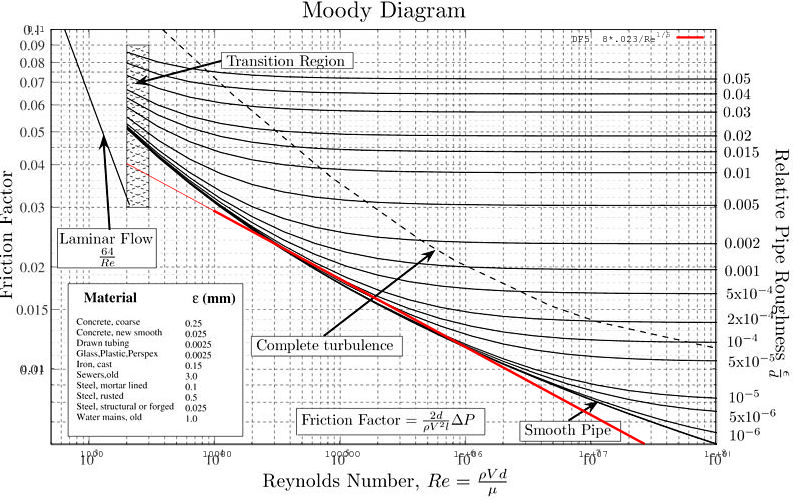### Boundary Layer

Lienhard and Lienhard write:

#### Turbulence near walls

... In a turbulent boundary layer, the gradients are very steep near the wall and weaker farther from the wall where the eddies are larger and turbulent mixing is more efficient. This is in contrast to the gradual variation of velocity and temperature in a laminar boundary layer, where heat and momentum are transferred by molecular diffusion rather than the vertical motion of vortices. In fact,the most important processes in turbulent convection occur very close to walls, perhaps within only a fraction of a millimeter. The outer part of the b.l. is less significant.

#### The viscous sublayer

... The [viscous] sublayer is on the order of tens to hundreds of micrometers thick, depending upon the fluid and the shear stress. Because turbulent mixing is ineffective in the sublayer, the sublayer is responsible for a major fraction of the thermal resistance of a turbulent boundary layer. Even a small wall roughness can disrupt this thin sublayer, causing a large decrease in the thermal resistance (but also a large increase in the wall shear stress).

Conversely, when the wall roughness is too small to disrupt the viscous sublayer, the convective surface conductance should be the same as for a smooth plate under the same conditions.

At the scale of the viscous sublayer, the inner radius curvature (being perpendicular to the flow) in a large diameter pipe should be indistinguishable from a flat plate. Because most of the convective thermal resistance occurs close to the surface of a pipe or plate, it is reasonable to expect the turbulent correlations for pipe and plate to be related.

### Forced Convection from a Rough Plate

Consider the similarity of Colburns's analogy (T8.1) to T8.9 and T8.11 for forced convection on plates:

 Nu = (f/2) Re Pr1/3 Colburn's analogy (T8.1) Laminar boundary layer:     Re < 5 × 105 Nu = 0.664 Re1/2 Pr1/3 Average value of h (T8.9) Turbulent boundary layer     beginning at leading edge:     All Re Nu = 0.037 Re4/5 Pr1/3 Average value of h (T8.11)

Combining equation T8.1 with each correlation and solving for Darcy friction factors yields:

 fD = 4⋅2⋅0.664 Re−1/2 DF9 fD = 4⋅2⋅0.037 Re−1/5 DF11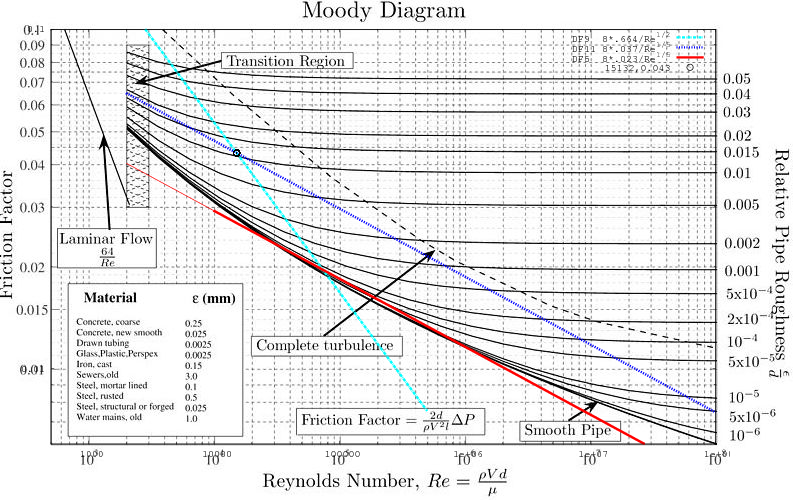DF11 in blue is parallel to DF5 in red because Re has the same exponent in both. DF9 in cyan looks like it may be parallel to the asymptote of the low Re end of the solutions of the Colebrook-White equation.

If D (and Re) in the Colebrook-White equation were scaled to move D5 to D11 then this scaled Colebrook-White equation would yield smooth flat plate correlations using the Colburn analogy.

Although it must have the correct relation to dimensions, the scale of characteristic-length is otherwise a matter of convention. The forced-convection correlations are usually monomials in Re and scale easily. With their characteristic-lengths being perpendicular, the geometry of pipes and flat plates are so different from each other that it is not unreasonable that scaling may be necessary in order to make correspondences between them.

While scaling the curve for ε=0 is straightforward, how should ε be scaled? The ratio between ε and DH does not inform how the ratio ε and L should scale. The ε scaling was determined from experiments described in Measurements of Convection From a Rectangular Plate.

Scaling the characteristic-length and Reynolds number by 9.5 in the Colebrook-White equation moves the friction curves to nestle into the flat plate asymptotics (DH=L/9.5). The mean-height-of-roughness ε is scaled so that the logarithm is of ε/L:1fP1/2 = −2 log10 ( εL + 9.5 ⋅ 2.51Re ⋅ fP1/2 ) SCW

The result of combining T8.1 with SCW can be expressed in terms of the unscaled Colebrook-White equation:

 Nu = 18 fD(Re/9.5, 3.7 ε/L) Re Pr1/3 Scaled-Colburn-analogy SCA

Correlation SCA transitions gradually between T8.9 and T8.11 around Re=17000.The graph to the right is of convective surface conductance versus (turbulent) fluid-speed for a smooth plate having length (in the direction of flow) of .305 m, 1 m, 10 m, and 100 m comparing T8.11 with the scaled-Colburn-analogy (SCA). The curves of each pair are close to each other. The scaled-Colburn-analogy curve is higher than the T8.11 curve for L=100 m because the Darcy friction factor levels out at high Reynolds numbers, raising the effective exponent from 4/5 (to 1).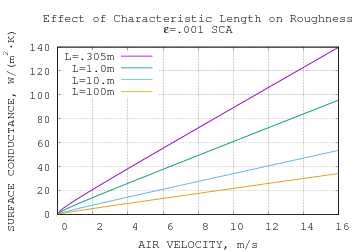The graph to the right shows that h becomes a nearly linear function of V when ε>0.

The graph below compares Fig. 24.1 curves (from Rowley et al) with the scaled Colburn analogy for the same roughness heights posited for forced convection in a duct. The curve for ε=.29 mm and stucco are the only match.### Colebrook-White Equation Asymptotes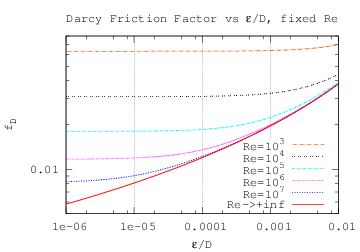At low Reynolds numbers fD has little sensitivity to relative roughness.

As Re tends to infinity, the Colebrook-White equation becomes (solid red in graph to the right):

 fD = 14 log10 ( ε / D3.7 )−2

The Colebrook-White equation assumes that the mean height of roughness, ε, is much smaller than the characteristic-length.As Re tends to infinity the scaled-Colebrook-White equation becomes:

 fP = 14 log10 ( εL )−2 SCWA

Note that the graph of the Colebrook-White equation and the scaled Colebrook-White equation have different abscissa ranges.

### Scaled Colburn Analogy Asymptote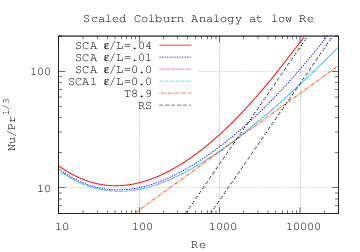Although the (friction factor) solutions of the Colebrook-White equation are monotonic as a function of Re, the Nusselt numbers resulting from their use in the Colburn analogy are not monotonic at low Reynolds numbers. These values are out of range for the Colburn analogy because the Colburn analogy assumes a laminar boundary layer depth dependent on the Reynolds-number [Prof. J. Lienhard, private communication]. So it behooves us to examine the correlation produced by asymptotics of the analogy. Combining the Colburn analogy (T8.1) with SCWA in the preceding section we find that for large Reynolds numbers over rough surfaces:

 Nu = 14 ⋅ 8 log10 ( εL )−2 Re Pr1/3 ≈ 0.031 Re Pr1/3log10( ε / L )2 0<ε≪L RS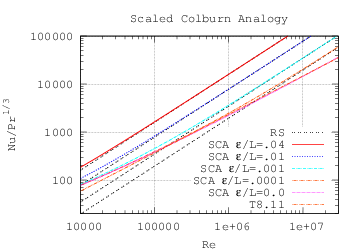The black dashed lines for RS in the graph to the right indeed tend to the scaled-Colburn-analogy correlations for rough plates at large Reynolds numbers.

Measurements from the Convection Machine below support the RS correlation over nearly an order of magnitude of Re range.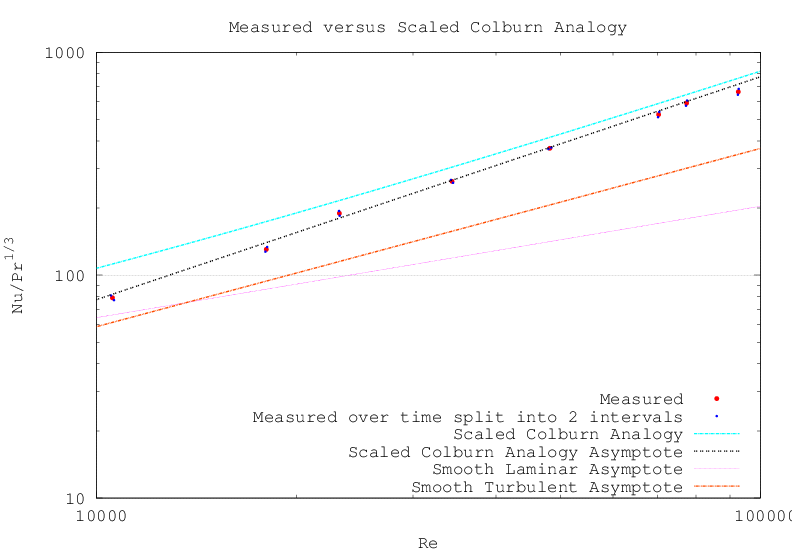Mixed Convection from a Rough Plate derives a Re upper bound for the scaled Colburn analogy asymptote.

 NuSCAA = Re Pr1/3 32 log10( ε / L )2 εLF < 0.085 Re1/5 SCAA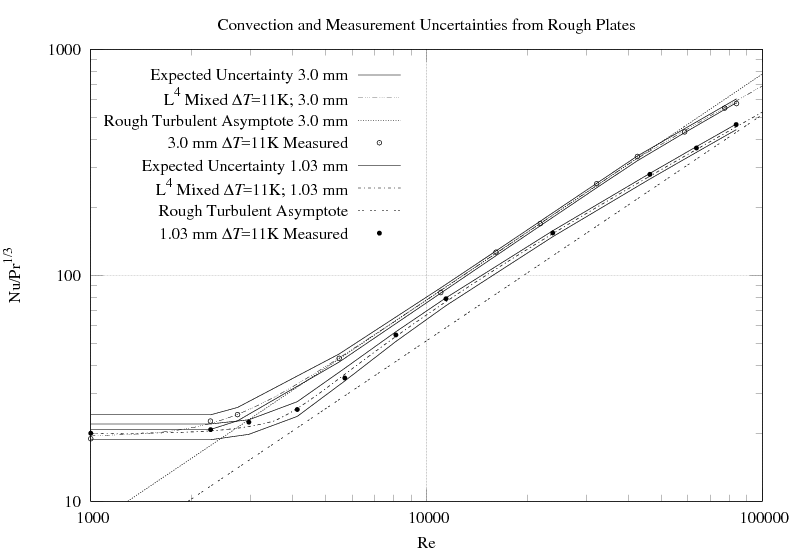In the graph above, the smooth-laminar asymptote is greater than part of the ε=3 mm curve, yet no laminar flow was observed in measurements. As the characteristic-length is scaled up, forced laminar convection grows more slowly than forced turbulent convection; forced laminar convection is not important for modeling roofs.

The plate in the Convection Machine will be modified to reduce its roughness in order to reveal the interaction between the scaled Colburn analogy asymptote and smooth-turbulent asymptote. Until those measurements are completed, I will assume that forced convection behaves as:

 hF = k⋅max(NuSCAA(Re), Nu8.11(Re))/LF

### Forced Convection Summary

Turbulent flow over both flat smooth plates and inside smooth pipes has Nu proportional to Re4/5. For the pipe, surface roughness which disrupts the viscous sublayer has Nu proportional to Re. Scaling the pipe correlations to match the plate results in correlation RS (which is proportional to Re) for rough plates. The overall convection is combined from correlations T8.11, and SCAA.

Proposed is the convective surface conductance for one side of a flat rectangular isothermal plate having mean height of roughness ε(≪L) with flow at velocity V parallel to one edge which is L long.

 Re = V⋅L / ν
 Nu8.11 = 0.037 Re4/5 Pr1/3 (T8.11) NuSCAA = Re Pr1/3 32 log10( ε / L )2 εLF < 0.085 Re1/5 SCAA

The forced-convection surface conductance is:

 hF = k⋅max(NuSCAA(Re), Nu8.11(Re))/LF

Experiments on the Convection Machine support correlation SCAA over the range 3000<Re<40000.

### Natural Convection from a Rough Plate

The Boundary Layer section asserts that laminar flow over a rough surface has the same convective surface conductance as laminar flow over a smooth plate. Note that when the mean-heigh-of-roughness is larger than the thickness of the viscous-sublayer (but still much less than Lc), the flow becomes turbulent and the assertion about laminar flow doesn't apply.

The boundary layer for downward convection (from a horizontal plate) is always laminar, its sole correlation having the Re exponent 1/5. It has the thickest boundary layer among orientations. If turbulence does appear, it will be in plumes from the edges of the plate, well away from the nearly all of the boundary layer (and not affect h).

The upward convection (from horizontal plate) measured by Clear et al was turbulent because of the large roof size. Their Rayleigh number of 1015 dwarfs the T9.8 upper bound of 1.5×109, yet they fit a coefficient of 1.01 to T9.8 for a horizontal roof (which wasn't smooth), but a significantly larger coefficient fitting T8.8 (local forced convection). They report the standard-error as 0.03 and 0.02, respectively. The coefficient of 1.01 indicates that natural convection from the rough roof was hardly different from that expected for a smooth roof.

Unlike plates in other orientations, buoyancy drives parcels of fluid warmed by an upward facing plate to change places with cooler fluid above them, directly away from the plate. Fluid flowing away from the plate would not experience friction with surface roughness. Is unheated fluid flow along the surface of plate affected by surface roughness? The streamlines in Fujii and Imura's figure 14(f) show most of the unheated fluid approaching the plate at angles between than 30° and 45° from horizontal. Without much fluid flowing along the plate's surface, it makes sense that turbulent upward convection would be unaffected by surface roughness.

The longer a parcel of fluid is in contact with the plate, the less additional heat is transferred to it. The most heat is transferred when the most fluid contacts the plate per unit time. Because the parcels immediately move away from the plate in T9.8 mode, it has the highest convective surface conductance among orientations.

Forced flow in a pipe experiences head (pressure) loss. The pressure being a force that acts through a distance (along the pipe), means that the flow does mechanical work on the system. The same should be true for flow along a vertical plate. The (convective) conversion of the heat difference to mechanical energy necessarily has poor efficiency. Thus the fluid velocity produced by natural convection must be much less than the forced fluid velocity producing the same surface conductance, all else being equal. The low turbulent shear stress resulting from low fluid speeds results in a thick viscus sublayer (described by Lienhard and Lienhard) where laminar flow dominates. As asserted by the Boundary Layer section, when the wall roughness is too small to disrupt the viscous sublayer, the convective surface conductance should be the same as for a smooth plate under the same conditions.

With the possible exception of very high Rayleigh-numbers on nearly vertical plates, natural convection from a rectangular plate is insensitive to surface roughness (0<ε≪L).

## Off-Axis Convection

Over flat plates, forced fluid flow is assumed to have straight, parallel streamlines. Thus convex plates with non-uniform characteristic-length can be analyzed by summing the results of the plate partitioned into strips which are parallel to the flow.

### Forced Off-Axis Convection

Generalizing the analysis at the end of Laminar Convection finds that the ratio between hd (diagonal) and hp (axis-aligned) is 2(1+E)/2/(1+E) where E is the exponent of Re. For forced turbulent convection (E=4/5), sensitivity to rotation of the square is less than 4%.Most roofs aren't square. Consider a S by rS rectangular plate with flow in the plane of the plate at angle φ from a length S side. For 0≤φ<π/2 and r≥tan φ, the strips which run between opposite rS sides have length K=S/cos φ. The base of the parallelogram is P=rSK⋅sin φ. The width of the parallelogram perpendicular to the flow is P⋅cos φ.

To find the average surface conductance for the whole plate, sum the product of the area of each strip and its average h (using its length as L), then divide by the total area rS2:

 ( h(K) K P cos φ + 2 K cos φ sin φ∫0 h( w cos φ sin φ ) w cos φ sin φ dw ) / (r S2)
 = .037 k Pr1/3 (V/ν)E ( KE P cos φ + 2(cos φ sin φ)E K cos φ sin φ∫0 wE dw ) / (r S2)
 = .037 k Pr1/3 (V/ν)E ( KE P cos φ + 2 K1+E1+E cos φ sin φ ) / (r S2)
 = .037 k Pr1/3 (V/ν)E (S/cos φ)E S/cos φ ( 1 + 1−E1+E ⋅ tan φr )
 = .037 k Pr1/3 (V/ν)4/5 (S/cos φ)-1/5 ( 1 + tan φ9 r )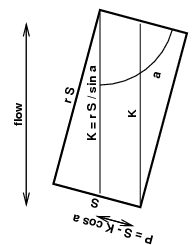When 0<r≤tan φ (and 0≤φ<π/2), the strips which run between opposite S sides have length K=rS/sin φ. The base of the parallelogram is P=SK⋅cos φ. The width of the parallelogram perpendicular to the flow is P⋅sin φ. The average surface conductance for the whole plate is:

 ( h(K) K P sin φ + 2 K cos φ sin φ∫0 h( w cos φ sin φ ) w cos φ sin φ dw ) / (r S2)
 = .037 k Pr1/3 (V/ν)E ( KE P sin φ + 2(cos φ sin φ)E K cos φ sin φ∫0 wE dw ) / (r S2)
 = .037 k Pr1/3 (V/ν)E ( KE P sin φ + 2 K1+E1+E cos φ sin φ ) / (r S2)
 = .037 k Pr1/3 (V/ν)E (r S/sin φ)E r S/sin φ ( 1 + 1−E1+E ⋅ rtan φ )
 = .037 k Pr1/3 (V/ν)4/5 (r S/sin φ)-1/5 ( 1 + r9 tan φ )

For every rectangle with r>1, there is an identical (but rotated 90°) rectangle with S'=rS and r'=1/r. Taking the geometric mean of the side lengths r1/2S as the characteristic length L allows these expressions to be written as the standard forced convection surface conductances (derived from T8.11 and T8.9) times rotation factors Ftur(rφ) and Flam(rφ):

 h = .037 k Pr1/3 (V/ν)4/5 L1/5 Ftur(r, φ) HTRR
 Ftur(r, φ) = |cos φ|1/5 r1/10 ( 1 + |tan φ|9 r ) r>|tan φ| FTRR ( |sin φ|1/5 / r1/10 ) ( 1 + r9 |tan φ| ) 0
 h = .664 k Pr1/3 (V/ν)1/2 L1/2 Flam(r, φ) HLRR
 Flam(r, φ) = |cos φ|1/2 r1/4 ( 1 + |tan φ|3 r ) r>|tan φ| FLRR ( |sin φ|1/2 / r1/4 ) ( 1 + r3 |tan φ| ) 0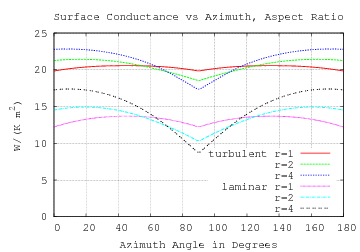To the right are shown the surface conductances for smooth (equal area) flat plates .35 m by .35 m, .5 m by .25 m, and .7 m by .175 m versus azimuth angle of the forced air flow (3.4 m/s at 21°C). At an azimuth of 0° the flow is parallel to a shortest side.

Both turbulent and laminar conductances are shown. The dips in the turbulent h will probably not be as sharp as shown due to the directional fluctuations in a turbulent flow.

The corresponding correlations are T8.11 and T8.9 times Ftur(rφ) and Flam(rφ) (with L = r1/2S):

 Nu = .037 Pr1/3 Re4/5 Ftur(r, φ) CTRR Nu = .664 Pr1/3 Re1/2 Flam(r, φ) CLRR

This is a mathematical derivation; I know of no in-plane off-axis forced-convection experiments.

That Ftur and Flam are independent of Re and L is a useful result. Ftur and Flam will be used to find h averaged over all azimuth angles in the next section.

The shape factor for the rough-surface correlation RS is more difficult to compute because it doesn't have a simple power dependency on L.

For r≥|tan φ|:

 K = S / |cos φ| P = r S−K |sin φ| = S (r − |tan φ|)

The average surface conductance for the whole plate is:

 hav = ( h(K) K P |cos φ| + 2 K |cos φ sin φ|∫0 h( w |cos φ sin φ| ) w |cos φ sin φ| dw ) / (r S2)
 = h(K) (1 − |tan φ| / r) + 2r S2 S |sin φ|∫0 h( w |cos φ sin φ| ) w |cos φ sin φ| dw

When 0<r≤|tan φ|:

 K = r S / |sin φ| P = S−K |cos φ| = S (1 − r / |tan φ|)

The average surface conductance for the whole plate is:

 hav = ( h(K) K P |sin φ| + 2 K |cos φ sin φ|∫0 h( w |cos φ sin φ| ) w |cos φ sin φ| dw ) / (r S2)
 = h(K) (1 − r / |tan φ|) + 2r S2 r S |cos φ|∫0 h( w |cos φ sin φ| ) w |cos φ sin φ| dw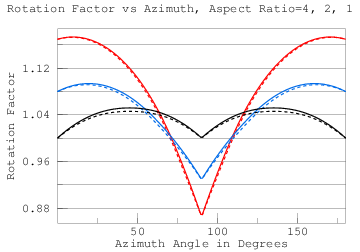The solid lines in the graph to the right show the rotation-factors for 1 m2 rectangular plates with ε=10-4 m and aspect-ratios of 1:1 (black), 2:1 (blue), and 4:1 (red).

### Effective Length

Clear et al give characteristic-length values of L=28.3 m and L*=10.2 m. The roof they measured was neither round nor rectangular, yet they find that meteorological wind direction doesn't correlate with turbulent convection from that flat horizontal surface:

None of the data showed any correlation with wind direction, so this was not included in any of the fits.

This could either be because surface condutivities of different wind directions on their roof are close in value; or because wind direction is so variable within their 15-minute sampling intervals that the variations mostly average out. The only information Clear et al give about the roof dimensions is area (2940 m2), perimeter (287 m), and mean distance of the perimeter from the measurement point (28.3 m which they use for the local forced L). Because they are working to match the measurements from an instrument cluster on a roof, they calculated local surface conductances. For application, average surface conductances are more useful.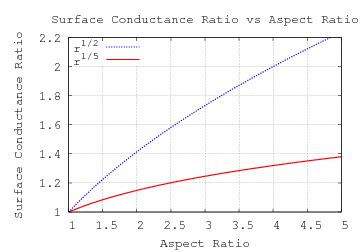A rectangle (their roof wasn't) with that area and perimeter would have an aspect-ratio of 4.8. The ratio of surface conductivities for a rectangle with aspect-ratio r along its two axis is:

 k ⋅ C ⋅ (V⋅S/ν)E Pr1/3 / S k ⋅ C ⋅ (V⋅r⋅S/ν)E Pr1/3 / (r⋅S) = r 1-E

In the turbulent case, an aspect-ratio of 2 leads to a surface conductance ratio of 1.15. That probably (and larger ratios certainly) would have been detected if wind directions were stable; so the independence of wind direction is probably due to its short-term variability.The Forced Off-Axis Convection section developed formulas Ftur and Flam for turbulent and laminar rotation factors which are functions of aspect-ratio and azimuth angle in the plane of a rectangular plate using the geometric mean of the sides as characteristic length. To the right is a graph of those rotation factors (numerically) averaged over 360° of flow direction versus aspect-ratio. The laminar curve Flam is solid black; turbulent Ftur is dashed black. The red curve is an approximation to Flam as a function of area A and perimeter P of the rectangle:

 0.615 + P 8.7 A1/2 = 0.615 + A1/2 8.7 L*

Expressing the shape factor in terms of general shape metrics may make extension to other convex polygons possible. A regular polygon approaches the circle as its number of sides increases. The shape factors for a circular disk under laminar and turbulent flow are shown as solid and dashed blue circles in the figure. The turbulent disk shape factor is 4% higher than its respective square plate having the same area; the laminar disk is 10% higher.Instead of multiplying h by a shape factor, Clear et al adjust the characteristic length to account for the effect of averaging over wind direction, calling it the effective length. The chart to the right shows factors which, when multiplied by the geometric mean of the sides (A1/2) produces the effective-length Leff. The effective-length shape factors are simply Ftur−5 and Flam−2

The spread between the shape factors grows from 3% at an aspect-ratio of 1:1 to 6% at an aspect-ratio of 10:1. Correlations with Re exponents between 1/2 and 4/5 will be contained in this band. Thus a single Leff (shown in red), which stays within those bounds will approximate Re exponents between 1/2 and 4/5 well.

 Leff = A1/2 / ( 0.615 + P 8.7 A1/2 )1/2 LEAAEffective-length greatly simplifies treatment of uniformly distributed flow direction. Can it do the same for Forced Off-Axis Convection? To the right are plots of laminar and turbulent off-axis rotation factors.Laminar and turbulent effective-lengths are very close, enabling a single formula for laminar and turbulent flows and use with the scaled-Colburn-analogy asymptote.

 Leff = A1/2 |cos φ|−1 r−1/2 ( 1 + |tan φ|3 r )−2 r>|tan φ| LERR A1/2 |sin φ|−1 r+1/2 ( 1 + r3 |tan φ| )−2 0The solid lines in the graph to the right show the rotation-factors for 1 m2 rectangular plates with ε=10-4 m and aspect-ratios of 1:1 (black), 2:1 (blue), and 4:1 (red) computed by integration. The dashed lines are the rotation factors computed using Leff.The graph to the right shows the rotation-factors by integration (solid) and using effective-length (dashed) for ε=10-3 m (higher) and ε=10-5 m (lower).

So the complexities of integrating RS turn out not to matter because the convective surface conductance of the rotated plate can easily be computed using effective-length LERR.

### Natural Off-Axis Convection

So far, the natural convective analysis has assumed a rectangular plate with at least one pair of horizontal edges. This section extends the analysis to horizontal and vertical rotated plates. Rotation of the plate out of the horizontal or vertical planes has already been covered.

The three modes of (convex flat plate) natural convection use different characteristic lengths, so they must be individually treated. The upward facing mode depends on L* (area/perimeter), which is already independent of rotation as evidenced by its working for a variety of convex shapes (Lloyd and Moran).

The downward convection mode is only active when the plate is horizontal. Its characteristic-length is half of the shortest side, which is also independent of orientation.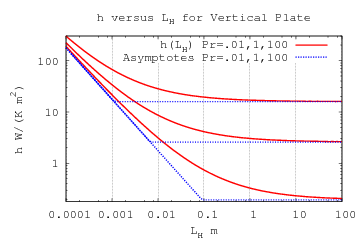The vertical plate convection mode corresponds to correlation T9.3 (1≤Ra≤1012):

 T9.3(Ra) = {.825 + .387 Ra1/6 [1+(.492/Pr)9/16]8/27 }2

Ra is proportional to L3. As a function of L, h9.3 is an L1/2-norm of 0.825 k/L and a constant term. The constant term is independent of L.A rotation factor for the 0.825 k/L term can derived analogously to FTRR and FLRR, but which reduces to a simpler form:

 F9.3(r, φ) = |cos φ| r1/2 + |sin φ| r−1/2 FVRR

The rotation factor for the constant term is 1.

The effective length Leff is simply A1/2/F9.3(rφ). It can be further reduced:

 Leff = LH LW |cos φ| LW + |sin φ| LH LVRR

The graph on the left compares h9.3(Leff) with h9.3(L)⋅F9.3, both with mean side lengths of 10-4 m. For side lengths greater than 1 m, h9.3 is much less sensitive to Leff and rotation. The graph on the right compares h9.3(Leff) with h9.3(L), both with mean side lengths of 1 m.

 A1/2=10-4 m A1/2=1 m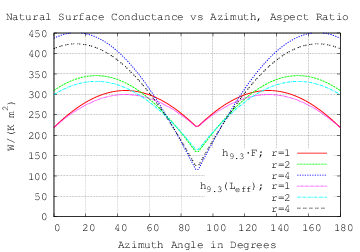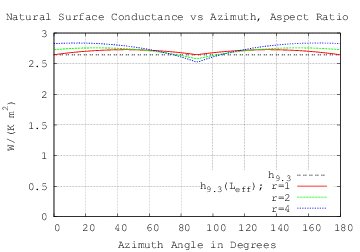## Mixed Convection

### Local Mixed Convection

In An Experimental Study of Mixed, Forced, and Free Convection Heat Transfer From a Horizontal Flat Plate to Air  X. A. Wang proposes local correlations for upward natural, mixed, and forced convection from a horizontal plate:

 Nuxf = 3+0.0253 Re0.8 for 0.068 Re2.2 > Gr (11) Nux = 2.7 ( Gr / Re2.2 )1/3 ( 3+0.0253 Re0.8 ) for 0.068 Re2.2 ≤ Gr ≤ 55.3 Re5/3 (12) Nuxe = 0.14 Gr1/3 for Gr > 55.3 Re5/3 (13)Fig.9 from the paper (recreated to the right) shows that the boundaries of the mixture zone (correlation 12) will cross. Equating the constraints finds that the intersection is at Re=2.86×105 and Gr=6.87×1010. The paper gives no guidance about how to treat Re and Gr values greater than this (which are in the roof range-of-interest).

The technique for finding average h in Laminar-Turbulent Progression only works when the correlation expression tends to zero as Re(x) goes to zero. The 3 added to 0.0253 Rex4/5 prevents the integral from converging. At high Re values, correlations 11 and 12 can be approximated:

 Nuxf ≈ 0.0253 Rex4/5 Nux ≈ 0.0683 Gr1/3 Rex1/15

The Wang correlations don't express a dependence on Pr. The working fluid is air with the plate temperature ranging from 19 K to 106 K hotter than ambient. The paper doesn't reveal the ambient temperature, and describes heaters but not coolers. Taking as ambient the mean temperature of Shanghai, 16.1°C, the Pr range for Wang's experiments is 0.715. to 0.723. Incorporating Pr back into correlations 11 and 13 yields formulas within 5% of T8.10 and T9.8:

 Nuxf ≈ 0.0282 Rex4/5 Pr1/3 Nuxe ≈ 0.156 Gr1/3 Pr1/3

Why can the local Nuxe be compared with the average Nu of T9.8? The dependence of Nuxe on x is linear; so its average h is the same as its (constant) local h.The measured data is shown only in Fig. 2, plotting local h versus distance from the leading edge. To the right, the values predicted by correlations 11, 12, and 13 are plotted in colors over Wang's graph. There are discontinuities at both transitions and, for x≥0.2 m, predicted h is significantly higher than measured.

Because Wang's (local) model doesn't work, trying to derive average h formulas from its divergent integrals would be pointless. Can a de novo analysis fit Wang's experiments?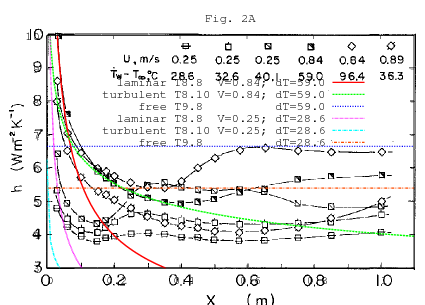The curves predicted by (forced laminar) T8.8, (forced turbulent) T8.10, and (natural turbulent) T9.8 for two cases are plotted on Fig. 2 to the right. For V=0.84, the transition from laminar to turbulent happens around x=0.075 m, at which Re=3548. This is much less than the Re=5×105 threshold given by Table 8. The low Re threshold could be due to a rough leading edge or turbulence in the impinging flow.

The two horizontal T9.8 lines are close in height to the right side of Wang's corresponding curves. But there were several problems: T9.8 should use L*=area/perimeter=0.05⋅L/(0.1+L), not the linear L that T8.8 and T8.10 use. In Wang's 0.1×1 m apparatus, L* ranges between 0 and 0.045 m. At the ΔT values from Fig.2A, Ra is bounded by 4.8×105, an order of magnitude below the range of applicability of T9.8.Forced convection is parametrized by distance from the leading edge because the flow controls the boundary layer near the edge. Natural upward convection from a horizontal plate has flow from all edges; that is why it uses L*. The heated strip in Wang's experiment is 10 times longer than it is wide. The forced flow at the leading edge will not affect most of that length, instead behaving as an infinite strip, L*=w/2. Unfortunately, the heated strip is flanked by insulated strips 0.075 m wide on each side. These reduce the natural convective flow across the edge of the heated strip, perhaps explaining why the measured h is lower than HHT* predicts, both for 0.1 m and 0.25 m widths. The graph to the right shows HHT* at two temperatures for (fully heated) widths of 0.1 m and 0.25 m.

### Correlations From Field Measurements

An Empirical Correlation for the Outside Convective Air Film Coefficient for Horizontal Roofs by R.D. Clear, L. Gartland and F.C. Winkelmann takes a quite different approach. The roofs of two commercial buildings were instrumented with thermal and meteorological sensors (at their centers) and sampled at 15 minute intervals for over a year. Because of the poor predictive power of the sky emissivity models, they exclued cloudy days. Along with exclusions for condensation and other reasons, they used less than 1/6 of the data collected.

Because of the size of the roofs, Ra at the measurement point always exceeded the range for laminar natural convection for ΔT > 0 and, in fact, often exceeded by a factor of 100 or more the recommended range of the equation for turbulent natural convection. Re is proportional to wind speed, and there were a substantial number of low wind speed points (< 0.1 m/s) that gave Re values that were nominally in the laminar flow region. However, the fits to the data were almost always better if the flow was assumed to be turbulent. In retrospect, it seems likely that any time natural convection is turbulent, then the mixed natural/forced convection should be turbulent also. All of our fits are based on turbulent flow at the measurement point for both the natural and forced convection conditions.C and D are the fitted constants in:

 f2(hn,hf ) ΔT = [ η C hn + D hf ] ΔT (4b)

where η is 1 for still air and tends to 0 as wind-speed increases:

 η = ln(1 + Grx / Rex2) 1 + ln(1 + Grx / Rex2)

Rex is the local Reynolds number because their wind-speed was measured at one point on the roof. The subscript of Grx implies that the Grashof Number is also local, but the correlations they used (T9.7 and T9.8) are for average surface conductance. It is possible that η requires adjustment for use with average Reynolds numbers.

Their fitted values are C=1.03 and D=1.66 with standard-error of 0.03 and 0.02, respectively.

With L*=10.2 m and ΔT=25 K, Ra is larger than 2×1012, exercising a scale of operation which is difficult to test in the laboratory. The two graphs below are of:

... a representative sample of data (July 1996, Davis) with cloudy days and condensation conditions removed, and corrected for conduction time lag between sensor location and roof surface.
We removed all data with ΔT < 0, i.e., roof temperature less than outside air temperature. This exclusion was, like the non-clear sky exclusion, due to our reliance on a Qsky estimation.
(In Figs. 8, 9 and 10, a small number of points with h < -20 or > 40 were not plotted to avoid losing detail in the remaining data.) During the day h generally increases during the morning to a mid-afternoon peak, then declines. This pattern reflects the ΔT and wind speed patterns at the site.
Figure 9 shows h vs. ΔT. The dependence on ΔT is fairly weak and we see the loss in precision as ΔT approaches zero.
Figure 10 shows h vs. wind speed. The dependence on wind speed is slightly sub-linear.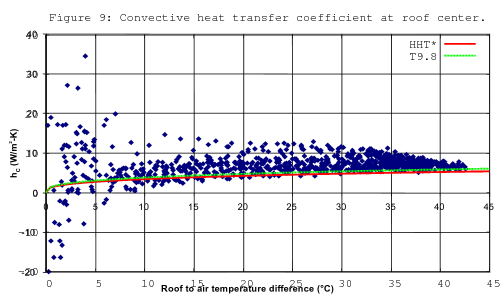Over Fig. 9 I have plotted the convective surface conductances predicted by correlations T9.8 and HHT* (natural convection above a hot horizontal plate). Above surface-to-air temperature differences of 20 K, the measured values are greater than or equal to those predicted by HHT*. This is consistent with the natural convection being aided by forced convection at low wind speeds. The forced convection contribution appears to diminish for larger temperature differences; this could be explained by the larger temperature differences being acheived only at low wind speeds (higher wind speeds cool the roof too much).

When the temperature difference is small:

... the long-wave radiative loss from the roof dominates: it is typically 10 times or more higher than the convective and conductive heat transfer. Under these conditions a small percentage error in Qsky can lead to large and systematic errors in hΔT ...

This could explain the flaring of h values below temperature differences of 10°C.

Because of the large Rayleigh numbers, HHT* is very close to T9.8. (Nu in) T9.8 is a linear function of L*; so h has no dependence on L*. Thus h at the center of the roof (where they measured it) should be the same as the average for the whole roof. The situation for forced convection is more complicated. The forced convection component at the center of the roof is local convection with characteristic length from the edge to center, rather than across the whole roof.

The characteristic length for forced convection is defined as the average distance from the roof perimeter to the heat transfer measurement point.

Their characteristic length for forced convection at the Davis site is 28.3 m. While the curve drawn on Fig. 9 is the curve for the average over the roof, the curves drawn on Fig. 10 are intended to reproduce the measurements taken at the center of the roof. The roof average will use a different L and involve T8.11 instead of T8.10 (whose ratio is 5:4).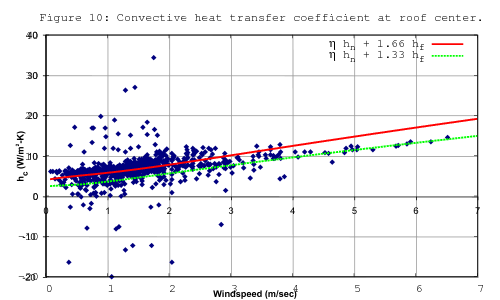Over Fig. 10 I have plotted f2(hn,hf) from equation (4b) for D values of 1.66 and 1.33 with ΔT values of 22 K and 4.4 K, respectively.

There are only 20 points at wind-speeds greater than 4 m/s. Considered in isolation they are a strong signal for a D value of 1.33. Because there are so few points at higher wind-speeds, one might conclude that h values at wind-speeds above 4 m/s are unimportant. But looking at the histogram of wind speeds for Honolulu shows that the yearly average wind speed is greater than 4 m/s at that locale.

In discarding 86% of the points, the authors raised the average surface-to-air-temperature-difference from 4.4 K to 22 K, a fivefold increase! The removal of low ΔT points introduced a large bias into the D value that the authors fit (1.66).

Can anything be salvaged from Fig. 10? While forced convection at low wind-speed aids natural convection, natural convection does not aid forced convection at higher wind-speeds. Thus the h values for wind-speeds greater than 4 m/s should be much less affected by the culling of low ΔT points than those at lower wind-speeds.

Clear et al model the effect of surface roughness as a scale factor of Nu (and h). The h versus V curves from Surface Roughness are similar enough to support fitting a scale factor. But the authors didn't measure the roughness of the roof; someone trying to apply their results has insufficient information for predicting h without reproducing their experiment to fit the hT8.10 coefficient D.

What about mixed convection from plates which are not level upward facing? Under the heading Limitations and Applicability, Clear et al write:

Our expressions for h are applicable in the following situations:
1. The roof is horizontal. However, it is probably safe to use the correlation for roof tilts up to about 20°. In no case should it be applied to vertical walls.
2. The roof is dry. The correlations should not be used when it is raining or when condensation is likely (surface temperature below dewpoint temperature).
3. The roof surface is flat and relatively unobstructed, i.e., at most a few percent of the roof area has protrusions like vents, roof-top equipment, etc.; the height of the roof parapet, if present, is only a few percent of the roof dimensions; and the roof surface is not in the wind shadow of another part of the building.

Caveat 1 rules out application of their work to vertical plates; caveat 2 rules out most downward convection.

The function η compares Gr=Ra/Pr and Re2. But in Natural Convection Summary, T9.3, HHT*, and Nu45 all use different characteristic-lengths, and Ra being proportional to L3 magnifies these differences. Adding to the difficulty of finding an effective Gr is that for downward convection from an inclined plate one of three characteristic-lengths applies depending on the h values computed with them. Furthermore, the Ra arguments to correlations T9.3 and HHT* are multiplied by cos θ and |sin θ|, respectively. Should η include these factors with Gr? The published convection literature provides no experimental basis for resolving any of these issues.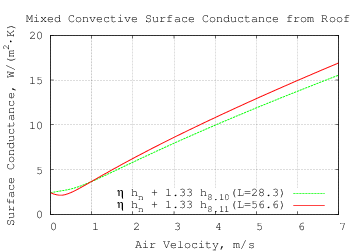Returning to horizontal plates, the graph to the right gives an expanded view of the green trace from Figure 10 above (local mixed convection). The red trace has double the forced characteric length L and uses T8.11 instead of T8.10 to model the average convective surface conductance from that roof. The dip at V=.25 m/s is due to the doubling of L (28.3 m to 56.6 m), not the switch from T8.10 to T8.11.This plot compares the red trace from the previous graph with scaled-Colburn-analogy curves for various surface roughness values (ε). Because of surface roughness, all but the lowest scaled-Colburn-analogy trace are nearly straight above 1 m/s; the lowest trace and the scaled T8.11 trace in red are proportional to Re4/5 above 1 m/s.

Consider the natural upward convection from a heated horizontal plate with a forced laminar flow parallel to the plate. Each (laminar) layer is shifted in the direction of the flow, the shift increasing with the layer's height above the plate. The relative shifting of the layers slightly increases the mixing of hotter fluid with cool fluid, increasing the heat transferred from plate to fluid. Therefore the surface conductance should be monotonically increasing with air velocity; so the η function, which produces a local minimum in the graphs above, doesn't appear to correctly model average surface conductance in laminar flows.

Forced turbulent flow erodes the laminar boundar layer, which also increases the surface conductance. Thus mixed convective surface conductance should increase monotonically with fluid speed.

### Mixed Convection from a Rough Plate

My article Mixed Convection from a Rough Plate develops a theory of convection for rough plates at any inclination and tests it against measurements from the Convection Machine. The Convection Machine plate with 3 mm of roughness is so rough that it doesn't explore the transition between turbulent flow from a smooth plate (Re4/5) and turbulent flow from the rough plate (linear Re). It will be modified to do so. In the meantime, I will assume that the two modes are in simple competition:

 Nu8.11 = 0.037 Re4/5 Pr1/3 (T8.11) NuSCAA = Re Pr1/3 32 log10( ε / L )2 εLF < 0.085 Re1/5 SCAA
 hF = k⋅max(NuSCAA(Re), Nu8.11(Re))/LF

Allowing for the plate to be rotated φ around its center adds more complication, which is detailed below. Reff is the effective-length for natural-convection from the plate.

### Mixed Convection Summary

Proposed is the complete convective surface conductance for one side of a flat LH by LW rectangular isothermal plate rotated φ around the center of the plate (in its plane) and inclined θ from vertical (θ<0 is facing upward) and with (forced) bulk fluid velocity is Vf at angle ψ from the normal projection of vertical in the plane of the plate.

 Pr = cp⋅μ/k

Shape Parameters

 A = LW LH r = LW / LH L* = A 2 (LW+LH) R = min(LH, LW)/2 Reff = LH LW |cos φ| LW + |sin φ| LH ‖x,y‖p = (xp+yp)1/p x ≥ 0; y ≥ 0; p > 0 θe = θ TS≥TF −θ TS

Natural Convection

 Ra* = β⋅|ΔT|⋅g⋅L*3 ν2 Pr |sin θe| RaV = β⋅|ΔT|⋅g⋅Reff3 ν2 Pr |cos θe| RaR = β⋅|ΔT|⋅g⋅R3 ν2 Pr |sin θe|
 NuHHT* = {0.671 + 0.370 Ra*1/6}2 −90°≤θe≤0° 1≤Ra*≤1012 Nu9.3 = {.825 + .387 RaV1/6 [1+(.492/Pr)9/16]8/27 }2 1≤RaV≤1012 Nu45 = 0.544 RaR1/5 [1+(0.785/Pr)3/5]1/3 0°≤θe≤+90°

The (forced) bulk fluid velocity is Vf at angle ψ from the normal projection of vertical in the plane of the plate.

The effective (characteristic) length for flow at angle ψ from the rectangle orientation is:

 Leff = A1/2 |cos ψ|−1 r−1/2 ( 1 + |tan ψ|3 r )−2 r>|tan ψ| LERR A1/2 |sin ψ|−1 r+1/2 ( 1 + r3 |tan ψ| )−2 0

Reynolds number for forced-convection:

 Re = V⋅Leff / ν

The forced component Nusselt number:

 Nu8.11 = 0.037 Re4/5 Pr1/3 (T8.11) NuSCAA = Re Pr1/3 32 log10( ε / Leff )2 εLeff < 0.085 Re1/5 SCAA
 NuF = max(NuSCAA(Re), Nu8.11(Re))

The mixed convective surface conductance is:

 M(hF, hN, ψ) = max(0, cos ψ) 4hF2hN2 hF4+4hN4 −min(0, cos ψ) 16hN16 hF16+16hN16
 B(hF, hN, ψ) = {[hF2+hN2]2−2hF2hN2M(hF, hN, ψ)}1/4
 h = k⋅max( B( NuF Leff , Nu45 R , ψ ) , ‖ NuV LV , NuF Leff ‖2) sin θe≥0 h = k⋅max( B( NuF Leff , NuHHT* L* , ψ ) , ‖ NuV LV , NuF Leff ‖2) sin θe≤0

The heat flow is h⋅A⋅ΔT.

## Application to Roofs

### Wind

A rotating cup anemometer at 10 m height is the standard wind-speed measuring instrument for the Automated Surface Observing System (ASOS); only the horizontal component of wind velocity is reported. There are formulas for estimating wind at lower heights. The important wind properties for convection are those near the roof surface. Wind speed and direction over the roof will be affected the shape and openings of the building; the most accurate modeling will require simulating airflow around the building.

For the low-pitch roofs examined here, the wind-speed, scaled for its height above terrain, should be a good estimate for the airspeed at the roof surface.

The range table for h predicts (using correlations RS and T8.11) that, for a 1 m square plate, 2 mm surface roughness can produce a forced convective surface conductance three times that of a smooth plate. In locations where ambient temperatures can exceed 45°C, a smooth roof may be preferable to a rough one in order to reduce wind-driven roof heating on very hot days.

### Flat Roofs

The simplest roofs are flat, either horizontal or slightly inclined. These are handled directly by the model. θ will be (slightly) greater than or equal to −90°.

Some horizontal flat roofs have low walls around their perimeters. This will reduce the forced convective surface conductance. As noted in the section Natural Convection from a Rough Plate, most of the inflow comes from above the plate, not along its surface. So the reduction of natural convective surface conductance may not be large.

Four Java methods in in Flat_roof.java calculate the mixed convection from a rough or smooth surfaced flat (rectangular) roof at given orientation, wind-direction and speed, temperature, air-pressure, and relative-humidity.

data-typenamereturn-unitsdescription
double`flatRoofConvect`Wheat flow
double`flatRoofHeatFlux`W/m2heat flux
double`flatRoofH`W/(K⋅m2)convective surface conductance
double`flatRoofHa`W/Kheat flow per degree of temperature difference

They all take the same arguments:

data-typeargumentunitsdescription
doubleh__mmlength
doublew__mmwidth
doubleeps__mmmean height of surface roughness
doublephi__deg°rotation around center in plane of roof
doubletheta__deg°inclination from vertical around width edge; negative is upward facing
doublepsi__deg°forced flow angle from width edge in plane of roof
doublev_f__m_per_sm/sforced bulk flow velocity
doublet_a__kKambient (bulk) air temperature
doublet_s__kKroof surface temperature
doublep__paPabulk air pressure
doublerhrelative humidity

### Peaked RoofsConsider a symmetrical peaked roof composed of two rectangular plates joind at the ridge. In the example to the right (top), each section has an 8 m span, a 2 m rise, and is 12 m long.

No rotation in the plane of the plates (which are not necessarily coplanar) is allowed, so φ=0. One of the constraints on the peaked roof model is that it should become identical to the Mixed Convection Summary model when θ=−90 (because the roof would then be flat).

−90≤θ<−60 is the inclination from vertical; the roof pitch is 90+θ (between 0° and 30°). LW is the length of the roof ridge and LH is the distance between the lower (parallel) edges of the roof.

 RH = LH |sin θ| A = LW RH r = LW / RH R = min(RH, LW)/2 Reff = RH/2 L* = A 2 (LW+RH) θe = θ TS≥TF −θ TS 0

A=LWLH/|sin θ| is the surface area of the roof and is the area by which h is multiplied.

For downward convection (roof cooler than air) Nu9.3 flow from the ridgeline will dominate Nu45 flow when the roof is not flat. The effective plate dimensions for Nu45 will be LW by LH when θ=−90 and are uncritical otherwise. The characteristic length for Nu9.3 flow is Reff=RH/2.

Natural Convection

 Ra* = β⋅|ΔT|⋅g⋅L*3 ν2 Pr |sin θe| RaV = β⋅|ΔT|⋅g⋅Reff3 ν2 Pr |cos θe| RaR = β⋅|ΔT|⋅g⋅R3 ν2 Pr
 NuHHT* = {0.671 + 0.370 Ra*1/6}2 −90°≤θe≤0° 1≤Ra*≤1012 Nu9.3 = {.825 + .387 RaV1/6 [1+(.492/Pr)9/16]8/27 }2 1≤RaV≤1012 Nu45 = 0.544 RaR1/5 [1+(0.785/Pr)3/5]1/3 0°≤θe≤+90°

The effective (characteristic) length for the mixed flow is independent of the sign of ψ. So in both cases it is:

 Leff = A1/2 |cos ψ|−1 r−1/2 ( 1 + |tan ψ|3 r )−2 r>|tan ψ| LERR A1/2 |sin ψ|−1 r+1/2 ( 1 + r3 |tan ψ| )−2 0

Reynolds number for forced-convection:

 Re = V⋅Leff / ν

The forced component Nusselt number:

 Nu8.11 = 0.037 Re4/5 Pr1/3 (T8.11) NuSCAA = Re Pr1/3 32 log10( ε / Leff )2 εLeff < 0.085 Re1/5 SCAA
 NuF = max(NuSCAA(Re), Nu8.11(Re))

The mixed convective surface conductance is:

 h = k⋅max(‖ Nu45 R , NuF Leff ‖4 , ‖ NuV LV , NuF Leff ‖2) sin θe≥0 h = k⋅max(‖ NuHHT* L* , NuF Leff ‖2 , ‖ NuV LV , NuF Leff ‖2) sin θe≤0

The heat flow is h⋅A⋅ΔT =h⋅ΔTLWLH/|sin θ|.

Four Java methods in in Peaked_roof.java calculate the mixed convection from a rough or smooth surfaced symmetrical peaked roof at given wind-direction and velocity, temperature, air-pressure, and relative-humidity.

data-typenamereturn-unitsdescription
double`peakedRoofConvect`Wheat flow
double`peakedRoofHeatFlux`W/m2heat flux
double`peakedRoofH`W/(K⋅m2)convective surface conductance
double`peakedRoofHa`W/Kheat flow per degree of temperature difference

They all take the same arguments:

data-typeargumentunitsdescription
doubleh__mmlength
doublew__mmwidth
doubleeps__mmmean height of surface roughness
doubletheta__deg°inclination from vertical; −90≤θ<−60
doublepsi__deg°forced flow angle from ridge in horizontal plane
doublev_f__m_per_sm/sforced bulk flow velocity
doublet_a__kKambient (bulk) air temperature
doublet_s__kKroof surface temperature
doublep__paPabulk air pressure
doublerhrelative humidity

The convecting surfaces of the peaked roof are convex when considered in combination. Two rectangular plates meeting at a lower height than their centers is not convex and their analysis is not addressed by current theory. For a valley roof, unless the joined edge is short compared with the other dimension, the T9.3 mode will be significantly reduced because of the extra distance air must travel to enter or exit near the joined edge.

### Roof PerformanceThe graph to the right compares heat flux from a flat level roof (black) with that from a symmetrical peaked roof with 30° pitch (red). The upper two traces are with 5 mm mean height of roughness; the lower two traces for a smooth surface. All are for roofs covering a 10 m by 10 m building with the roof 5°C hotter than ambient.

The wind direction is diagonal across the roof. At wind-speeds greater than 4 m/s the surface roughness has greater effect on heat-flow than the roof configuration.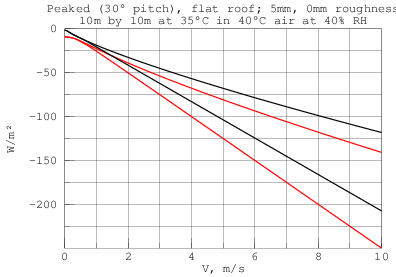The graph to the right shows the heat flux from the same roofs, but with the roofs 5°C cooler than ambient. The peaked roof's Nu45 mode is overwhelmed by T9.3 in still air, so its downward natural convection does not get as close to 0 as the flat plate does.

## Conclusion

A complete mixed single-phase convection model has been presented for flat rectangular plates. Its novel features are:

• natural convection from a rectangular plate in any orientation,

• a correlation for forced convection over a rough flat surface,

• correspondence between forced convection and the vertical-plate mode of natural convection,

• vector sum of forced-equivalent velocity and forced velocity vectors,

• competition between convective modes, and

• L4-norm combination of natural convective modes.

## Future Work

### Flat Convex Plates

The model could be extended to arbitrary convex shapes by extending each of the convective component modes to arbitrary shapes. The shape-factor for both the forced and vertical-plate modes can be computed by integration as was done in Forced Off-Axis Convection and Natural Off-Axis Convection.

The upward convective mode (HHT*) is a function of L*, which is defined for all flat convex shapes. Lloyd and Moran find the formula accurate for a variety of convex shapes.

The challenge is finding the characteristic-length for downward convection from a horizontal plate. Downward convection from a rectangular plate self-organizes into two rollers parallel to a longest edge and has characteristic-length, R, which is half of the shorter edge. Schulenberg gives a separate correlation (47) for downward convection from a disk which has the same exponents as correlation (45) for an infinite strip. Through appropriate choice of characteristic-length R, can these two correlations be unified?

### Convection with Phase-Change

Convection in fluids which can undergo phase changes is of great practical interest in the case of water-vapor condensation on roofs. While there is a body of theory and experiment dealing with condensation from a pure phase-change fluid (eg. steam), there is less available dealing with condensation from a mixture of condensing and non-condensing gases (eg. air).

### Experiments

#### Measure the dependence of Nu/Pr1/3 on Re and relative roughness 0<ε≪L.We know that for small Reynolds number flow over low surface roughness, the convective surface conductance is close to that of a smooth plate. So only strong turbulent flow over a rough surface needs to be measured. Not needing laminar flow simplifies the wind tunnel requirements considerably.

The table below shows the dimensionless quantities that the scaled-Colburn-analogy-asymptote model predicts for plates with relative roughnesses of 0.01, 0.005, and 0 (smooth).

ε/L Re Nu/Pr1/3 Re Nu/Pr1/3 Re Nu/Pr1/3 0.0100 2.00×104 1.56×102 4.00×104 3.12×102 8.00×104 6.25×102 0.0050 2.00×104 1.18×102 4.00×104 2.36×102 8.00×104 4.72×102 0.0000 2.00×104 1.02×102 4.00×104 1.78×102 8.00×104 3.09×102

This measurement has been performed by the Convection Machine for ε/L=0.01 between Re=5000 and Re=100000; the results appear to confirm RST.

#### Determine if surface roughness affects turbulent natural convection of a vertical rectangular plate.

The reasoning that natural vertical convection is insensitive to surface roughness might not hold at high Rayleigh numbers.

The plate described in Convection Measurements is not large enough to reach turbulent natural convection in air with a practical temperature difference (<50K).

#### Measure the dependence of natural surface conductance h on orientation of a rectangular plate.

Because the natural convection arising from the the plate described in Convection Measurements is expected to be laminar, it can be used to do this measurement. However, the apparatus designed for testing the higher heat transfer rates of forced convection will suffer from long settling times measuring natural convection.

#### Measure the surface conductance h of a plate (in various orientations) at natural-to-forced transition flows.

This would require stable laminar low-speed flow in the wind tunnel, which can be difficult to achieve and measure accurately.

## Bibliography

1. 1997 ASHRAE Handbook-Fundamentals (SI),
American Society of Heating, Refrigerating and Air-Conditioning Engineers, Inc.,
Atlanta, US, 1997.

2. 2009 ASHRAE Fundamentals Handbook (SI)
American Society of Heating Refrigerating and Air-conditioning Engineers Inc.,
ISBN: 978-1-931862-70-7 (I-P); 978-1-931862-71-4 (SI)
ISSN: 1523-7222 (I-P); 1523-7230 (SI)

3. The Electronics Handbook, Second Edition,
Editor: Jerry C. Whitaker,
Technical Press, Morgan Hill, California, USA, 2005
ISBN: 9780849318894

4. Kyle A. Brucker, Joseph Majdalani,
Effective thermal conductivity of common geometric shapes,
International Journal of Heat nd Mass Transfer,
Volume 48, Issues 23-24, November 2005, Pages 4779-4796,
ISSN 0017-9310, DOI: 10.1016/j.ijheatmasstransfer.2005.05.007.

5. R. J. Goldstein, E. M. Sparrow, D. C. Jones,
Natural convection mass transfer adjacent to horizontal plates,
International Journal of Heat and Mass Transfer,
Volume 16, Issue 5, May 1973, Pages 1025-1035,
ISSN 0017-9310, DOI: 10.1016/0017-9310(73)90041-0.

6. J. R. Lloyd and W. R. Moran,
Natural Convection Adjacent to Horizontal Surface of Various Planforms,
J. Heat Transfer 96, 443-447 (1974),
DOI:10.1115/1.3450224

7. Stuart W. Churchill, Humbert H. S. Chu,
Correlating equations for laminar and turbulent free convection from a vertical plate,
International Journal of Heat and Mass Transfer,
Volume 18, Issue 11, November 1975, Pages 1323-1329,
ISSN 0017-9310,
DOI: 10.1016/0017-9310(75)90243-4

8. Tetsu Fujii, Hideaki Imura,
Natural-convection heat transfer from a plate with arbitrary inclination,
International Journal of Heat and Mass Transfer,
Volume 15, Issue 4, April 1972, Pages 755-764,
IN5-IN6, 765-767, ISSN 0017-9310, DOI: 10.1016/0017-9310(72)90118-4.

9. T. Schulenberg,
Natural convection heat transfer below downward facing horizontal surfaces,
International Journal of Heat and Mass Transfer,
Volume 28, Issue 2, February 1985, Pages 467-477,
ISSN 0017-9310, DOI: 10.1016/0017-9310(85)90080-8.

10. X. A. Wang,
An Experimental Study of Mixed, Forced, and Free Convection Heat Transfer From a Horizontal Flat Plate to Air,
J. Heat Transfer,
Volume 104, Issue 1, February 1982, Pages 139–144,
DOI: 10.1115/1.3245040.

11. Automated Surface Observing System (ASOS) User's Guide,
Department of Defense
United States Navy
March 1998
http://www.nws.noaa.gov/asos/

12. E. M. Sparrow and K. K. Tien,
Forced Convection Heat Transfer at an Inclined and Yawed Square Plate—Application to Solar Collectors
J. Heat Transfer,
Volume 99, November 1977, Issue 4, 507 (6 pages)
DOI: 10.1115/1.3450734.

13. C. P. Kothandaraman,
Fundamentals of Heat and Mass Transfer,
Third Edition, 2006,
New Age International (P) Limited, Publishers, New Delhi

14. R.D. Clear, L. Gartland and F.C. Winkelmann,
An Empirical Correlation for the Outside Convective Air Film Coefficient for Horizontal Roofs,
Environmental Energy Technologies Division,
Lawrence Berkeley National Laboratory,
LBNL-47275 January 2001

15. S Beck and R Collins, University of Sheffield,
File:Moody diagram.jpg,
Wikipedia, the free encyclopedia, 2008,

16. Rowley, F.B., Algren, A.B., and Blackshaw, J.L.,
Surface Conductances as Affected by Air Velocity, Temperature and Character of Surface,
ASHVE Trans. 36, p 429 (1930).

17. John H. Lienhard IV and John H. Lienhard V,
A heat transfer textbook, 4th edition, 2011,
Phlogiston Press, Cambridge, Massachusetts, US
TJ260.L445

18. Churchill, Stuart W.
A comprehensive correlating equation for forced convection from flat plates,
AIChE Journal, Volume 22, Issue 2, 1975,
DOI: 10.1002/aic.690220207

19. C. F. Colebrook and C. M. White
Experiments with Fluid Friction in Roughened Pipes,
Proc. R. Soc. Lond. A 1937 161, 367-381,
DOI: 10.1098/rspa.1937.0150

20. Moody, L. F.,
Friction factors for pipe flow,
Transactions of the ASME 66 (8): 671–684 (1944)

21. Latif M. Jiji,
Heat Convection,
Springer-Verlag Berlin Heidelberg,
ISBN 978-3-642-02970-7

22. H. T. Lin, W. S. Yu, C. C. Chen,
Comprehensive correlations for laminar mixed convection on vertical and horizontal flat plates,
Heat and Mass Transfer, Vol. 25, No. 6. (1 November 1990), pp. 353-359.,
doi:10.1007/BF01811559 Key: citeulike:8824378

23. P. T. Tsilingiris,
Thermophysical and transport properties of humid air at temperature range between 0 and 100°C,
Energy Conversion and Management,
Volume 49, Issue 5, May 2008, Pages 1098-1110,
ISSN 0196-8904, http://dx.doi.org/10.1016/j.enconman.2007.09.015.

24. Z. K. Morvay, D. D. Gvozdenac,
Applied Industrial Energy and Environmental Management,
Toolbox 6: Thermodynamic and Transport Properties of Moist Air,
John Wiley & Sons, Ltd

25. K. Kadoya, N. Matsunaga, and A. Nagashima,
Viscosity and Thermal Conductivity of Dry Air in the Gaseous Phase,
J. Phys. Chem. Ref. Data 14, 947 (1985),
http://dx.doi.org/10.1063/1.555744 (24 pages)

26. A. Wexler,
Vapor pressure formulation for water in range 0 to 100°C. A revision (1976),
J. Res. Nat. Bur. Stand. Sec. A: Phys. Ch., Vol. 80A, Nos. 5&6, p. 775,
http://archive.org/details/jresv80An5-6p775